# ML Aggarwal Solutions for Class 10 Maths Chapter 3 Shares and Dividends

ML Aggarwal Solutions For Class 10 Maths Chapter 3 Shares and Dividends are provided here for students to practice and prepare for their exams. All these solutions have stepwise explanations, which aim at a student’s better understanding. The ML Aggarwal Solutions available at BYJU’S will help students to clear their doubts and get full marks for the questions from this chapter, in their final exams. Students can easily master the concepts of Shares and Dividends. Shares are units of ownership interest in a corporation or financial asset that provide for an equal distribution in any profits, if any are declared, in the form of dividends. A sum of money paid regularly (typically annually) by a company to its shareholders out of its profits is called dividends. These solutions are really helpful to get a better understanding of the concepts of shares. ML Aggarwal Solutions for Class 10 can be downloaded in PDF format. These solutions help to develop confidence among students who are preparing for exams. ML Aggarwal Class 10 Maths Solutions of Shares and Dividends present on this page provide solutions to the questions discussed in this chapter.

## PDF Solution for ML Aggarwal Class 10 Maths Chapter 3 :-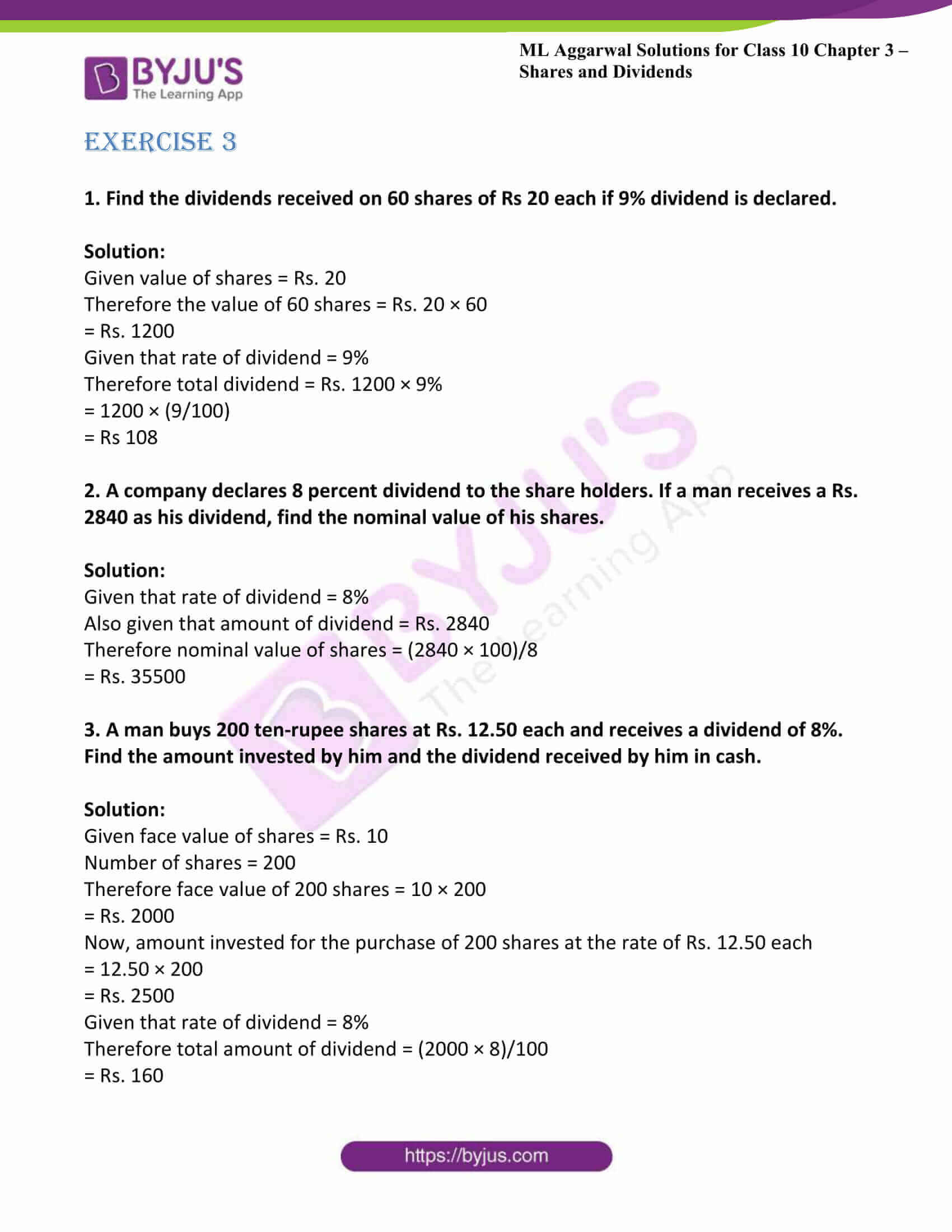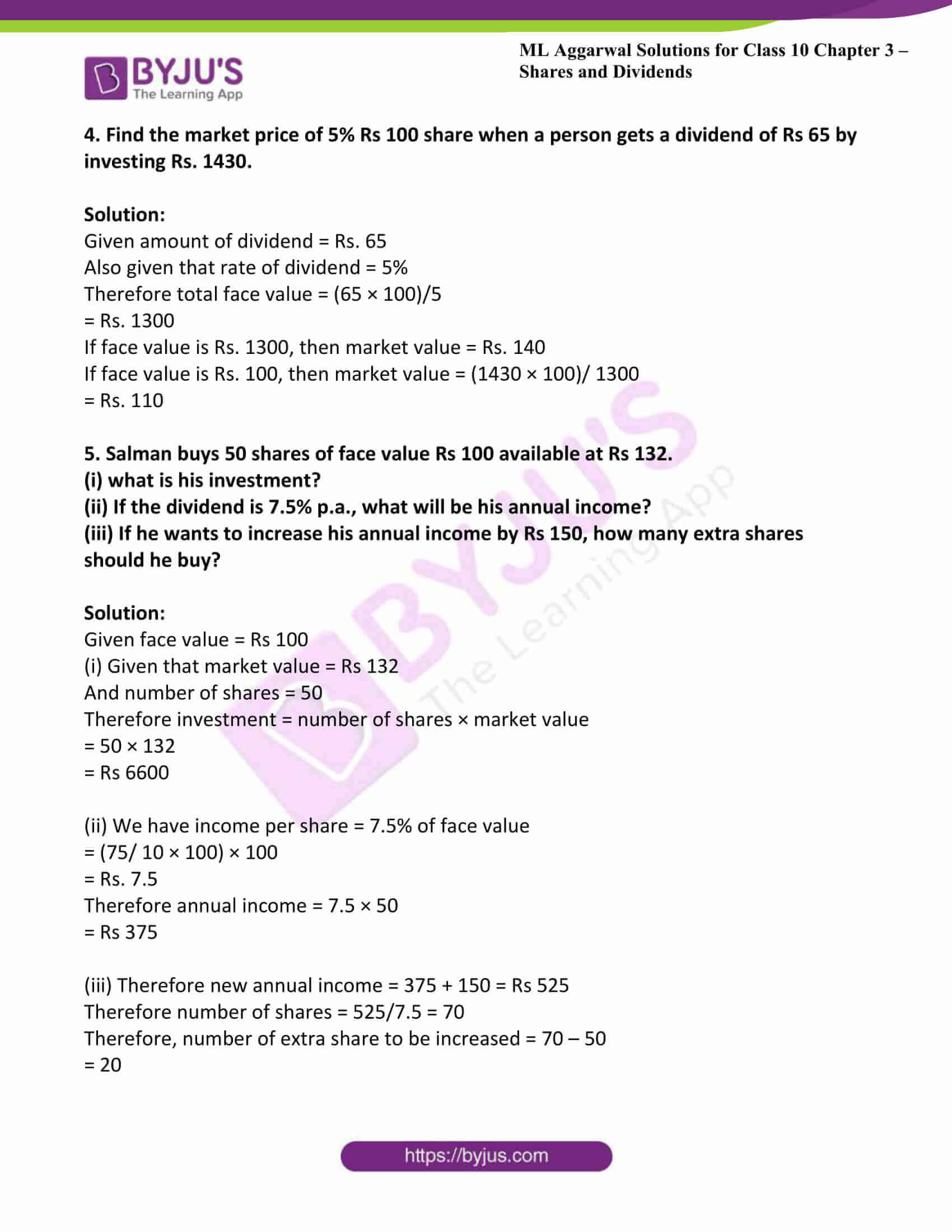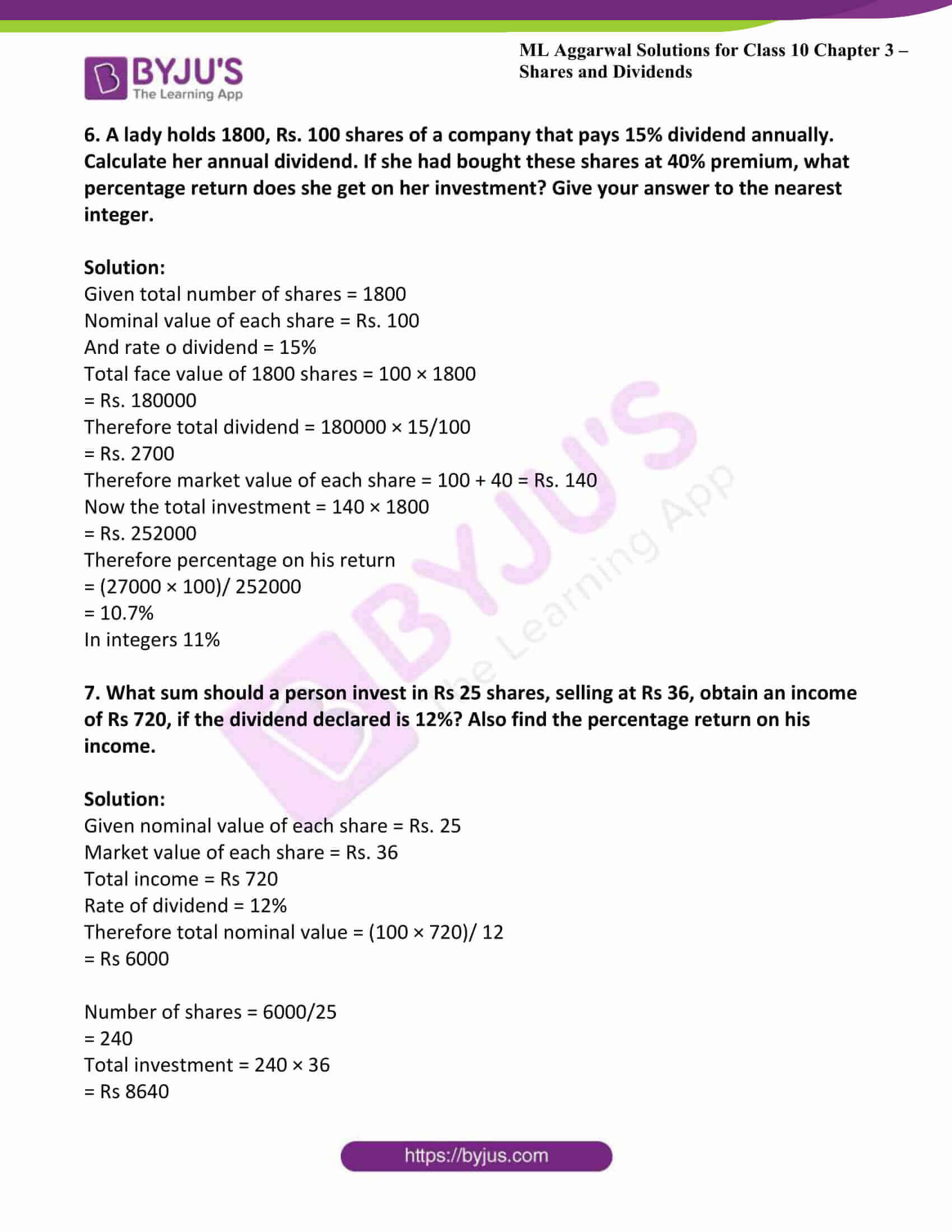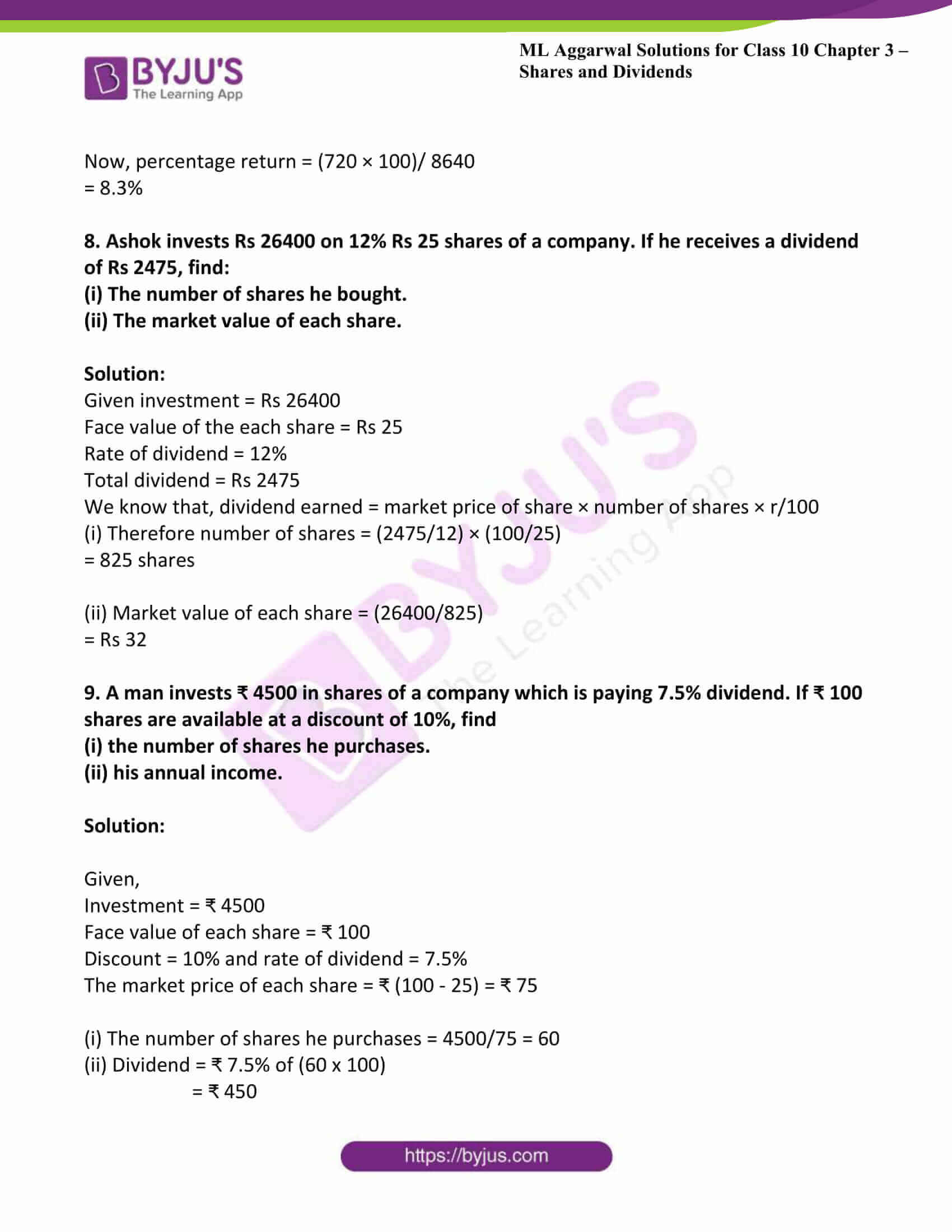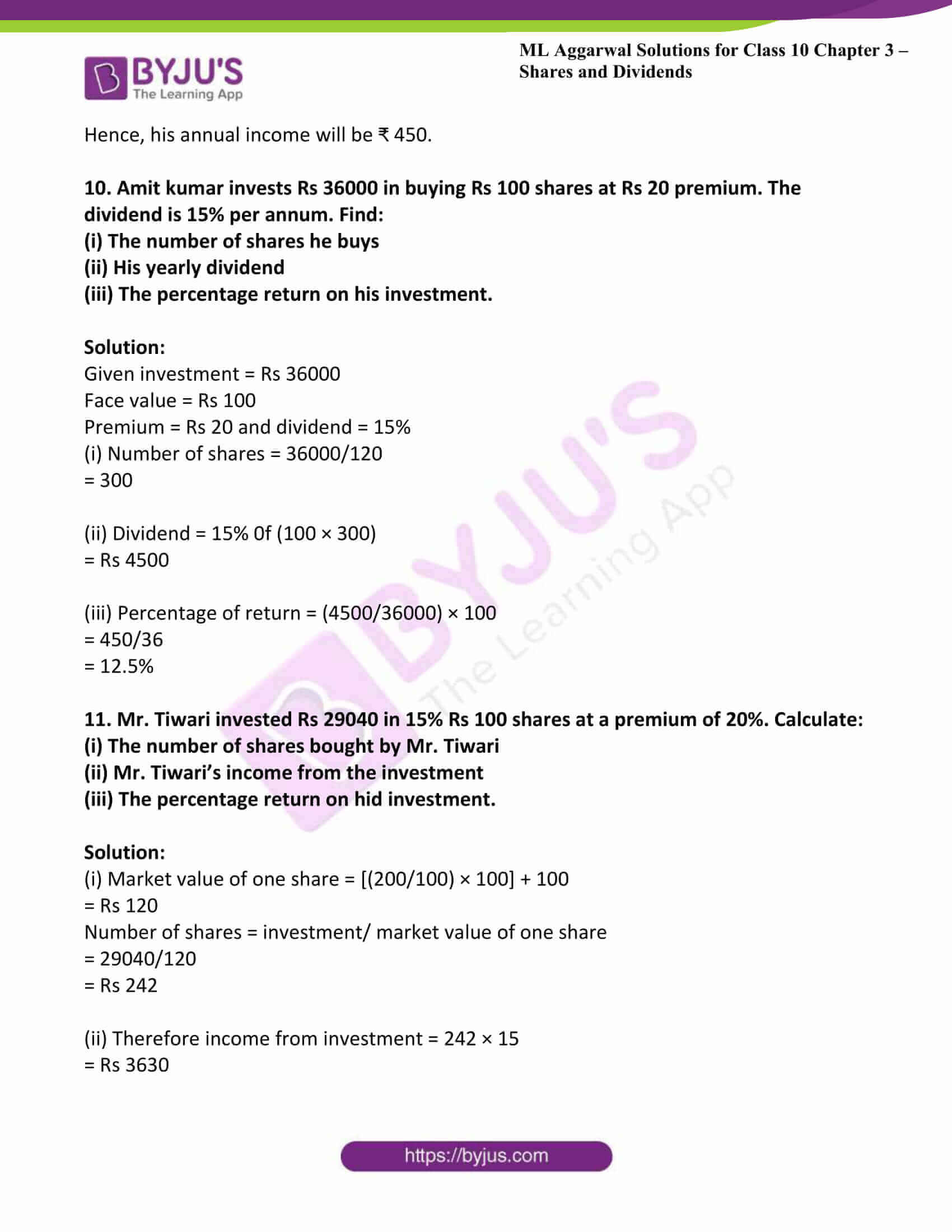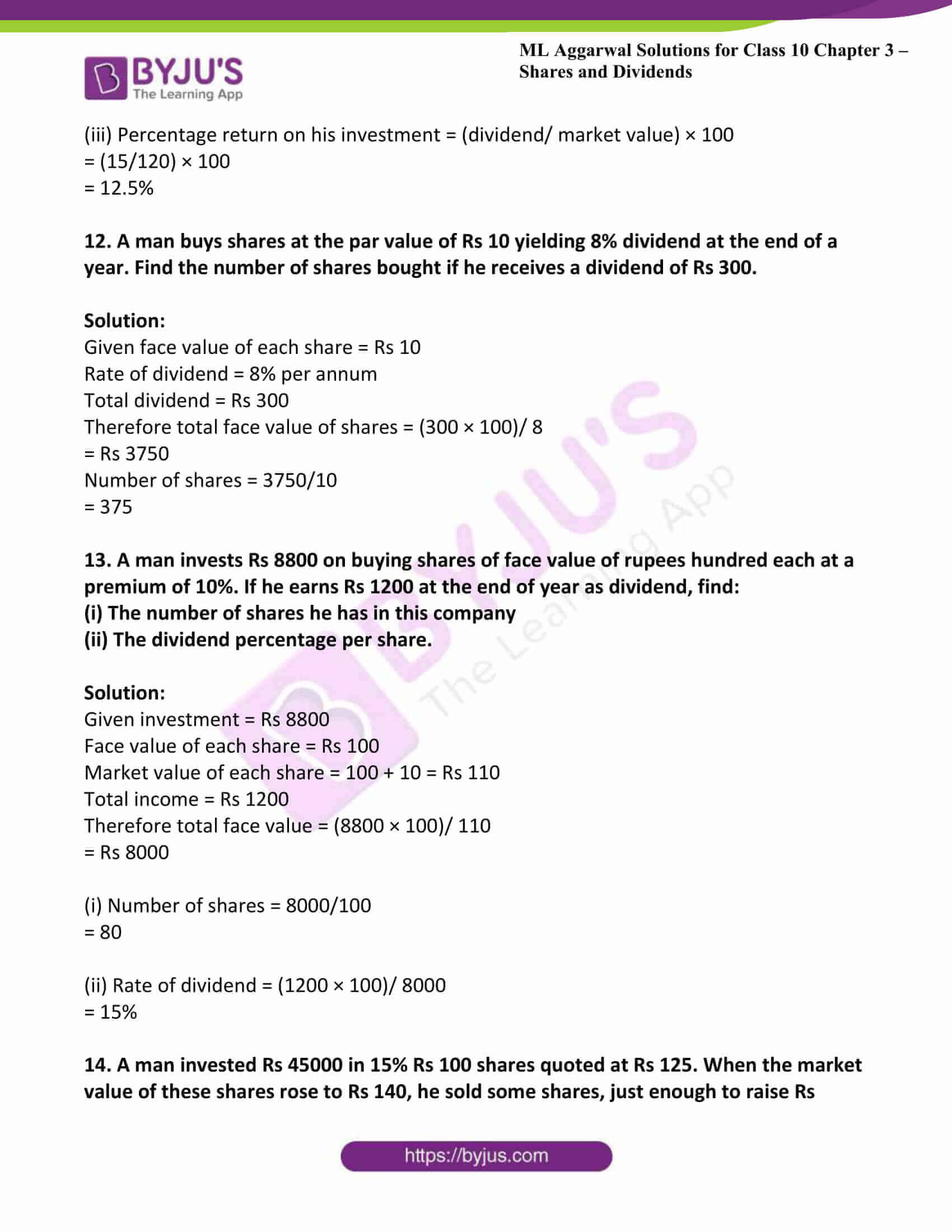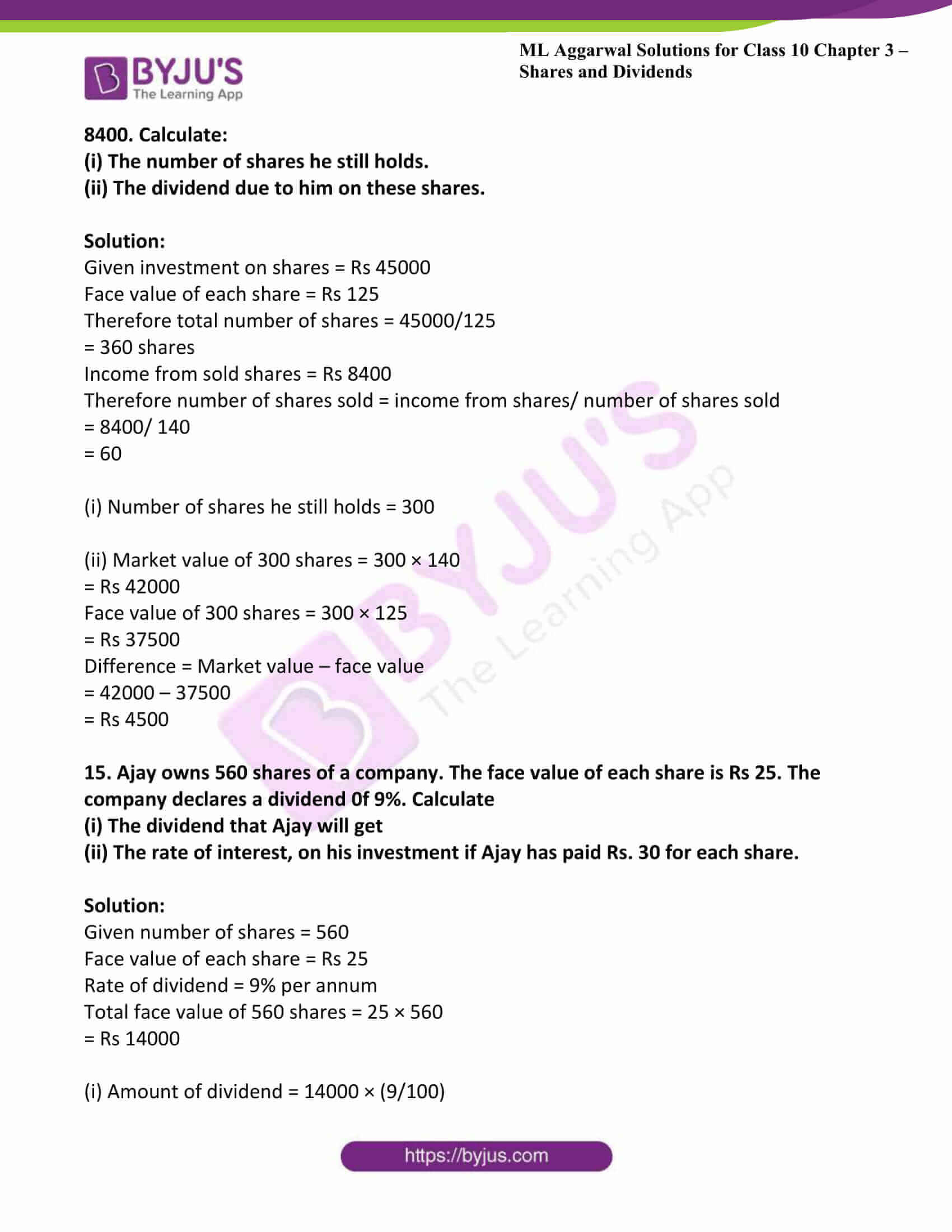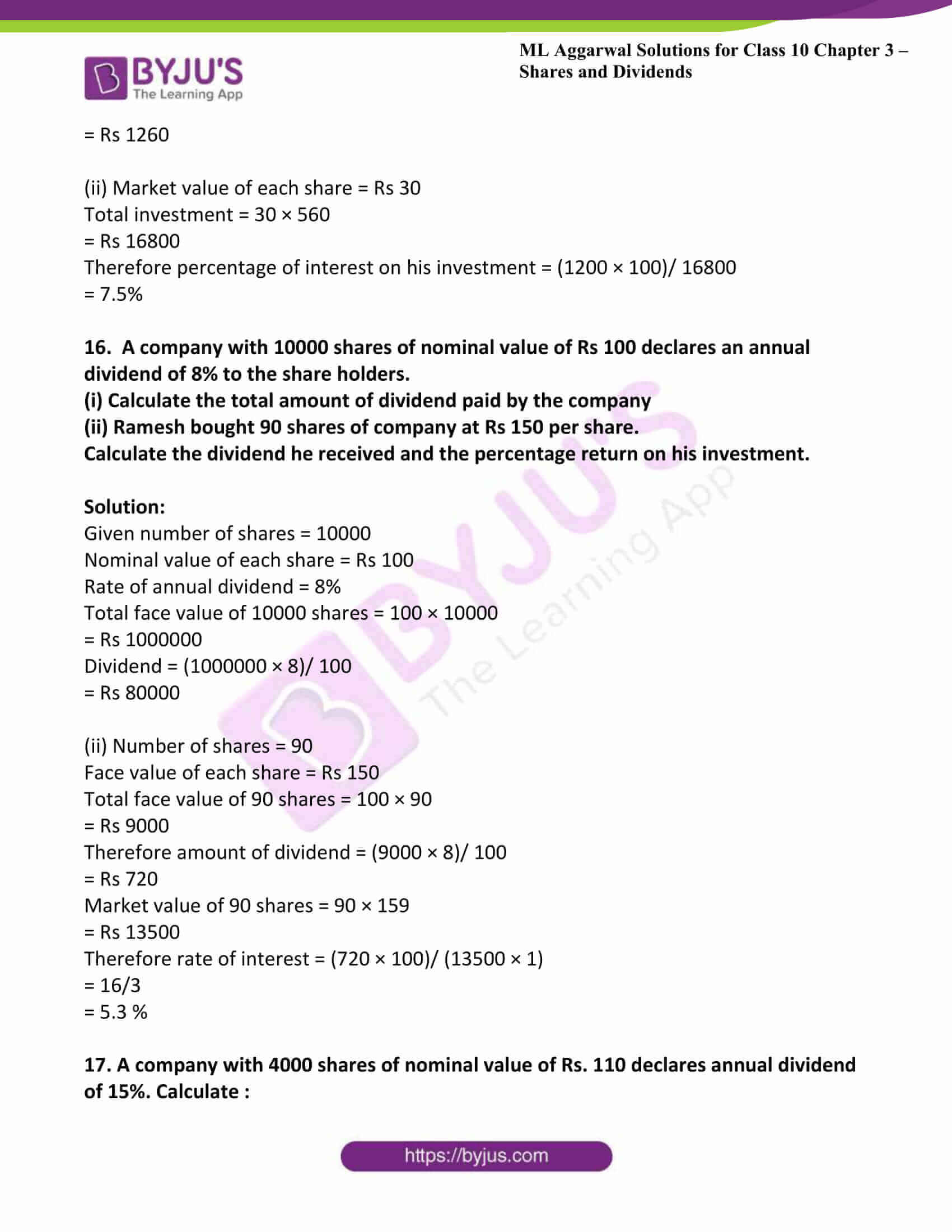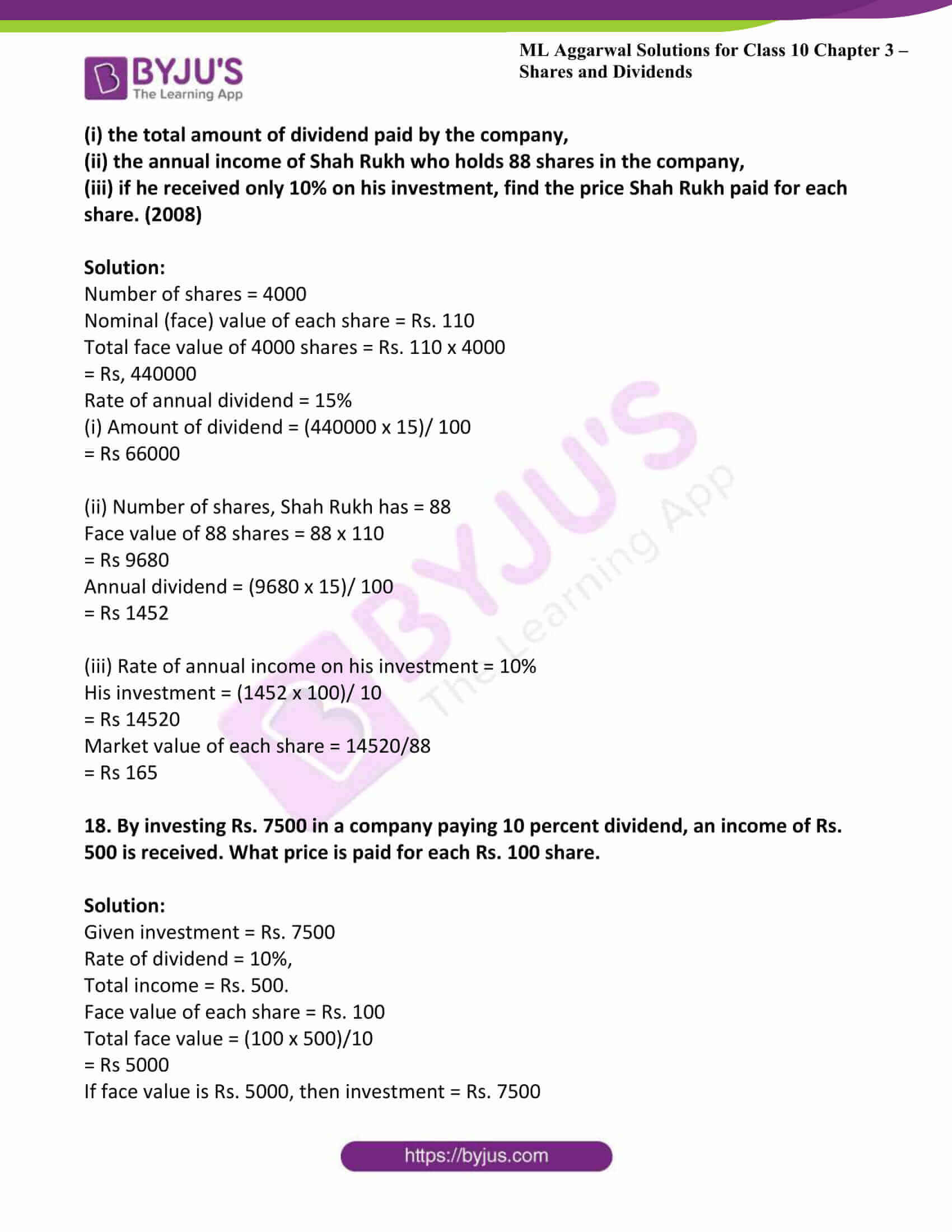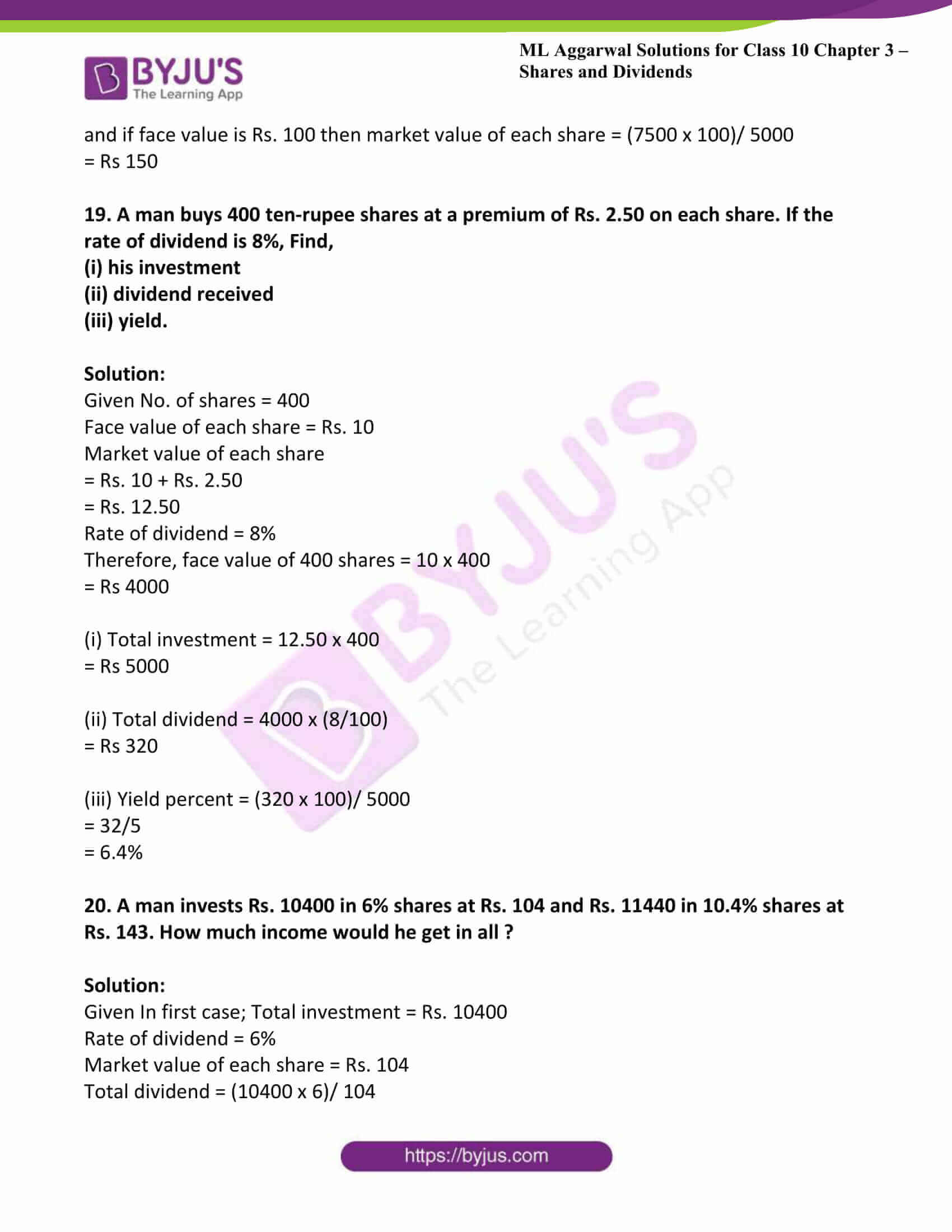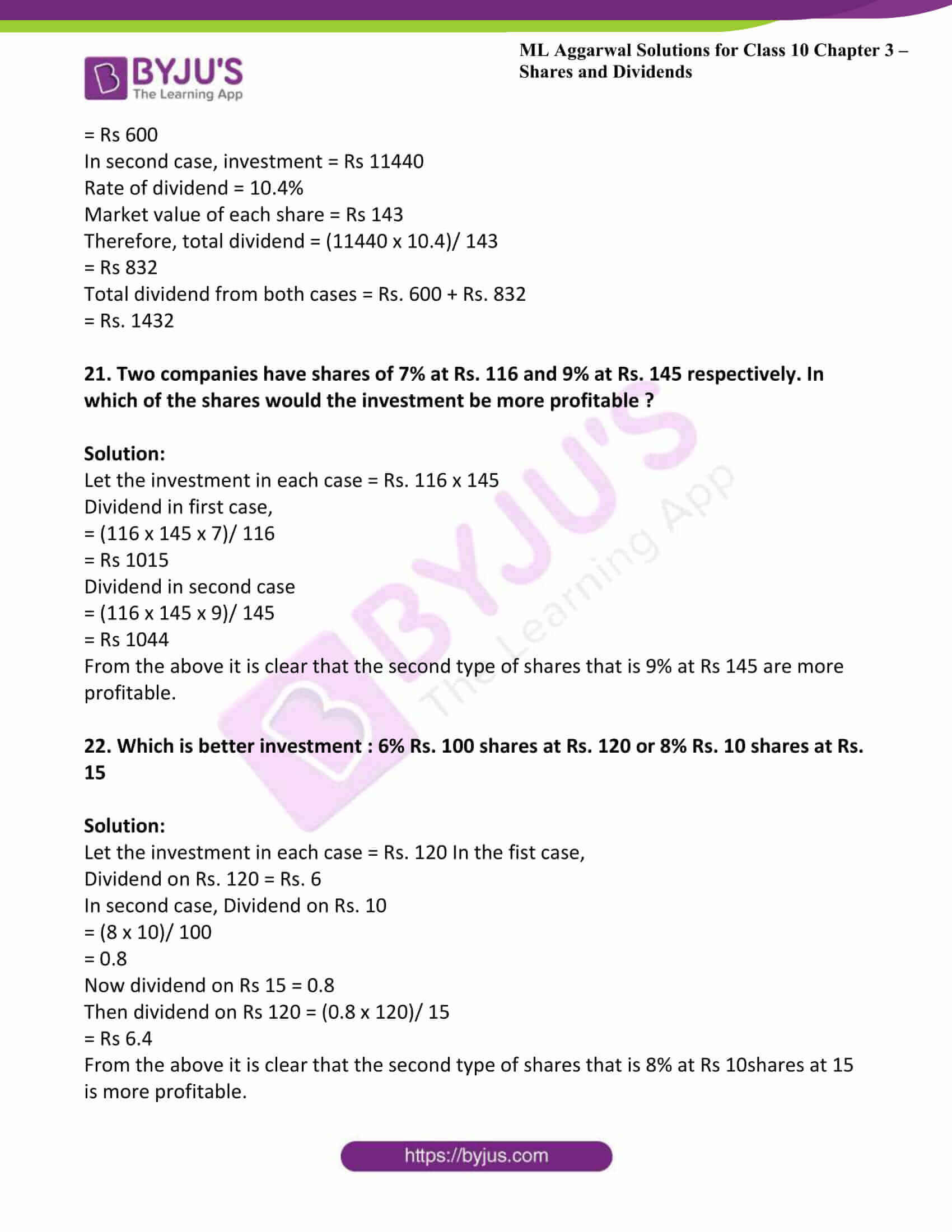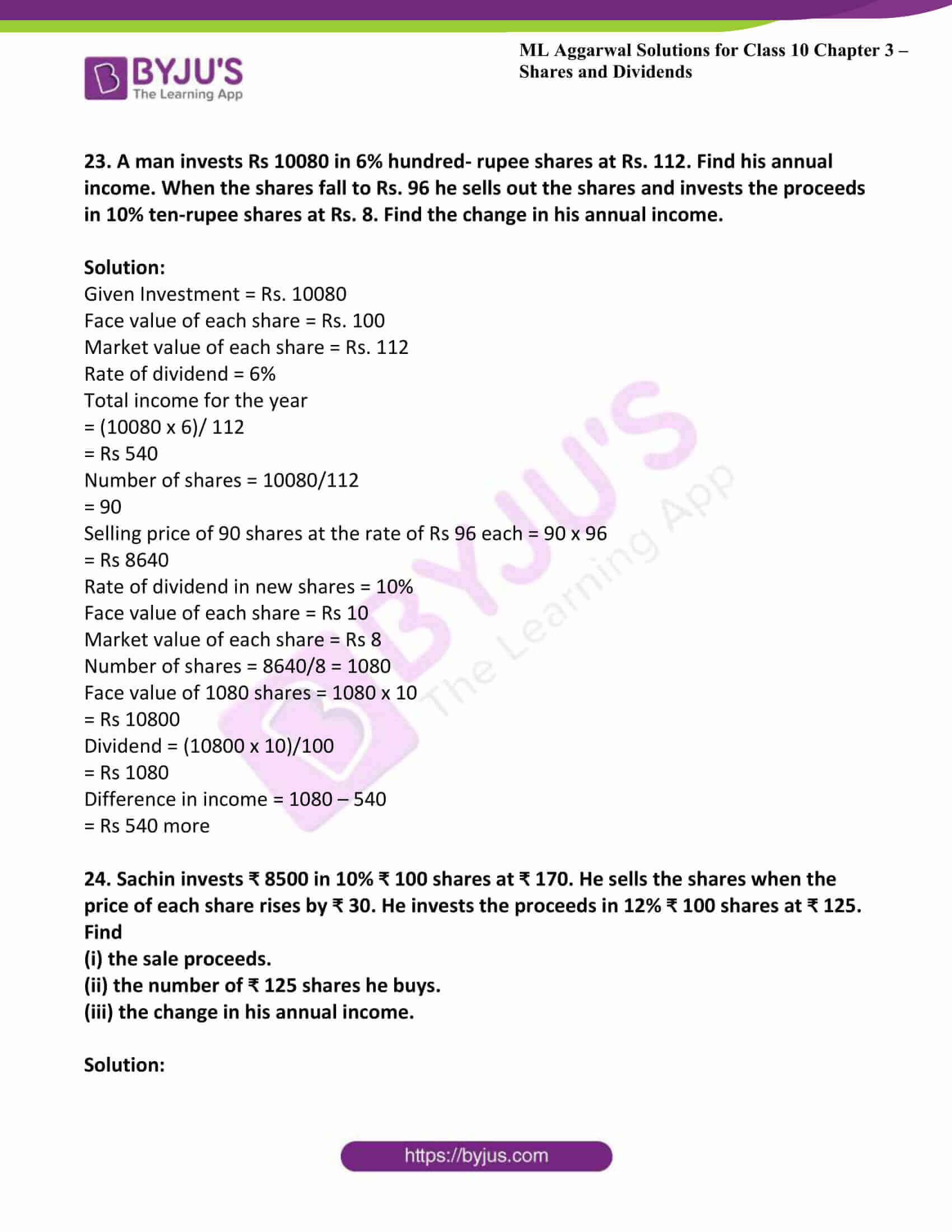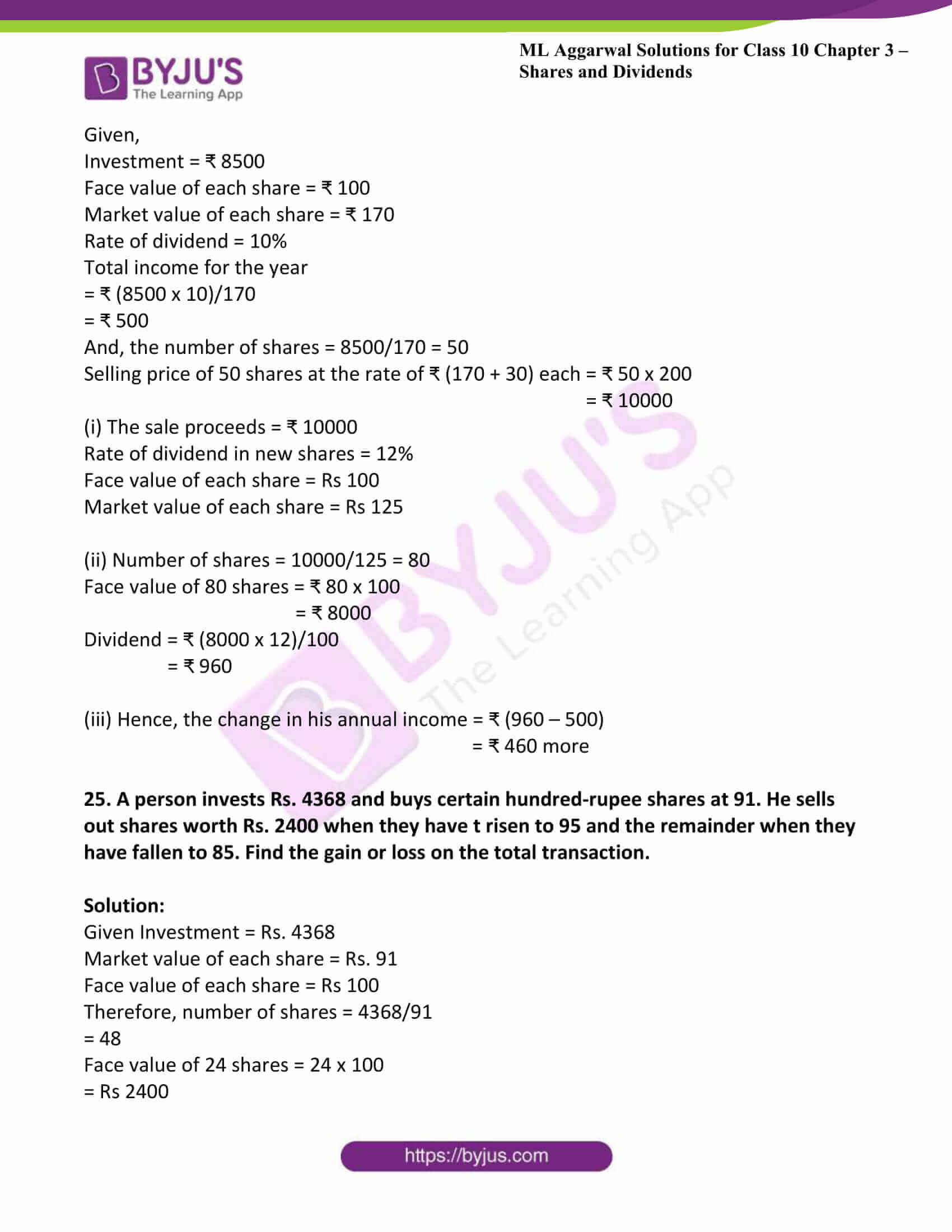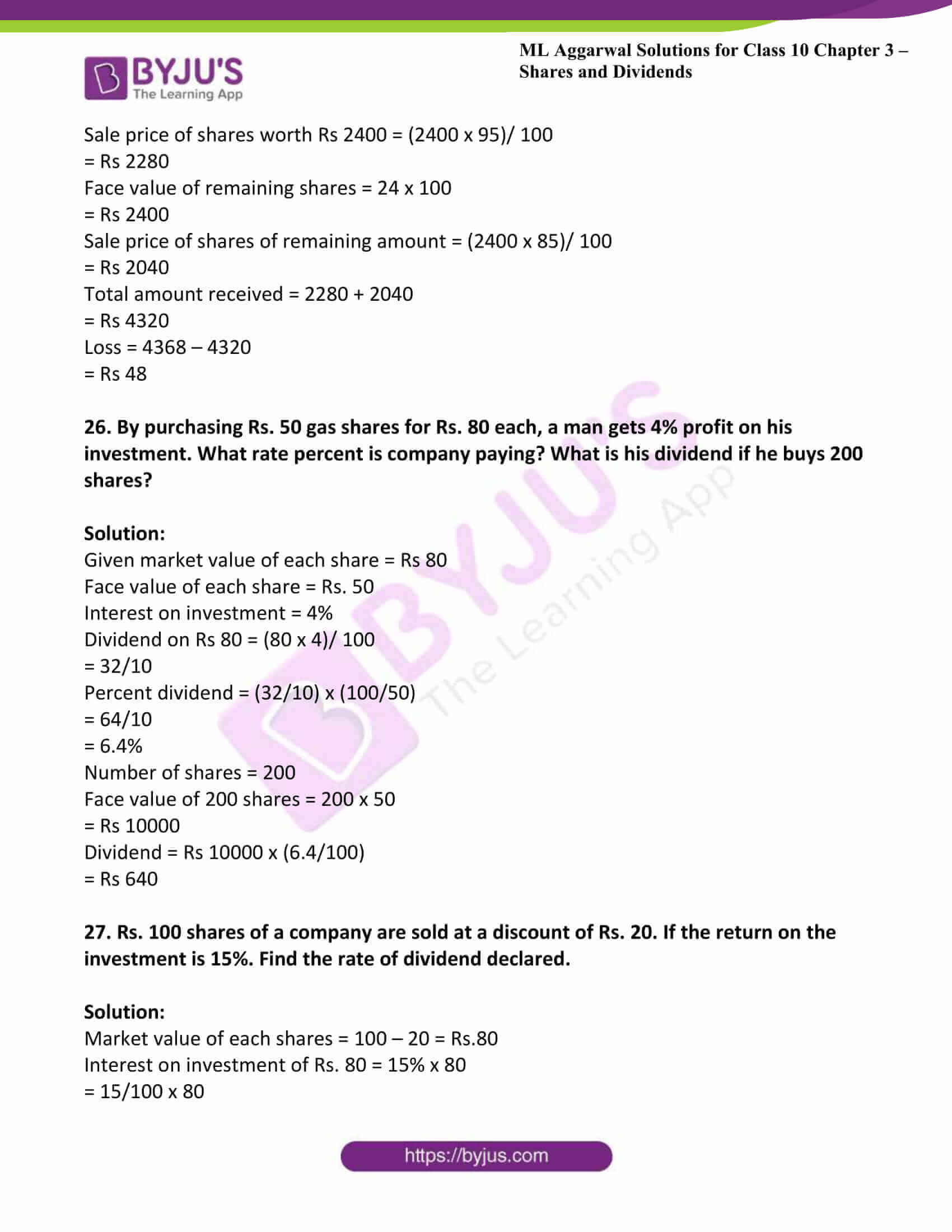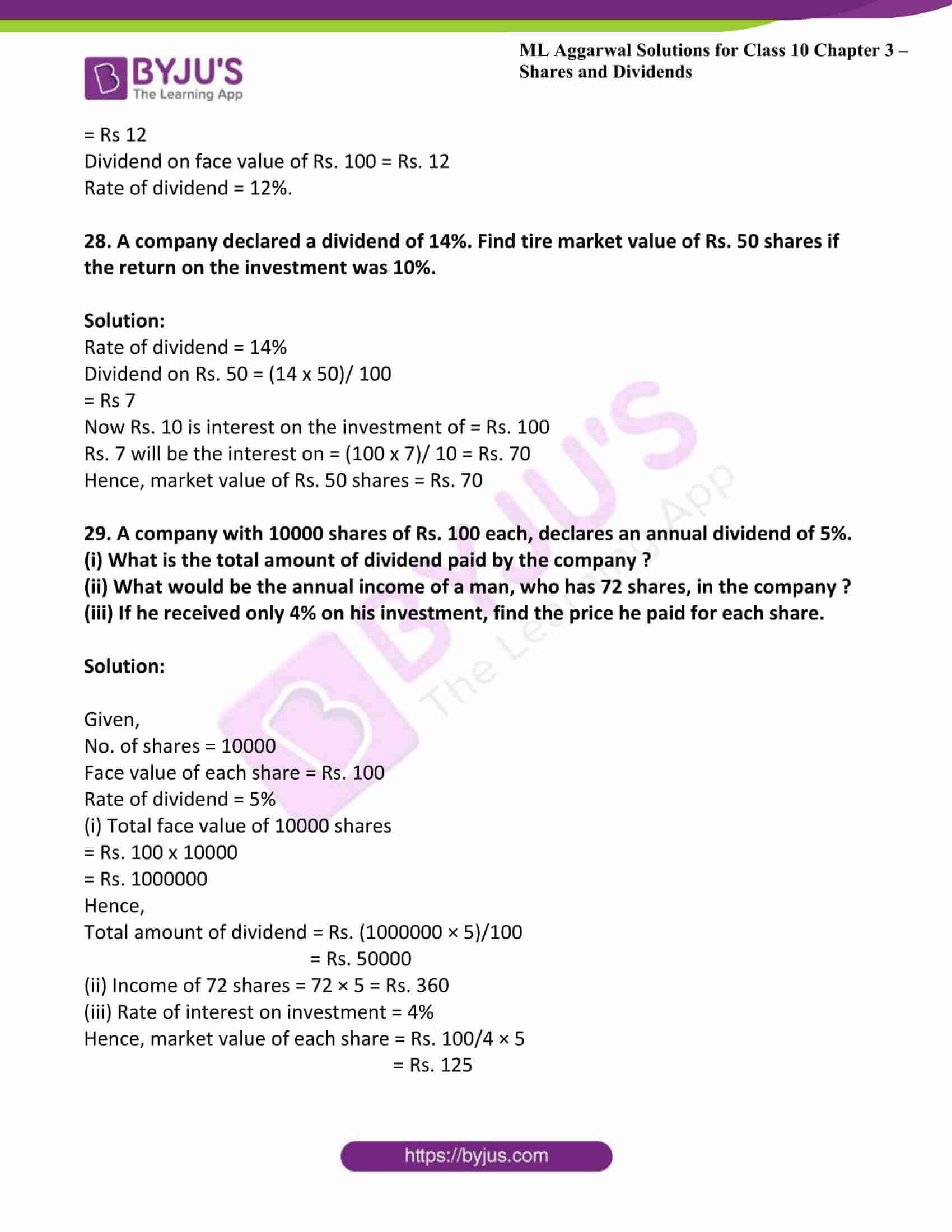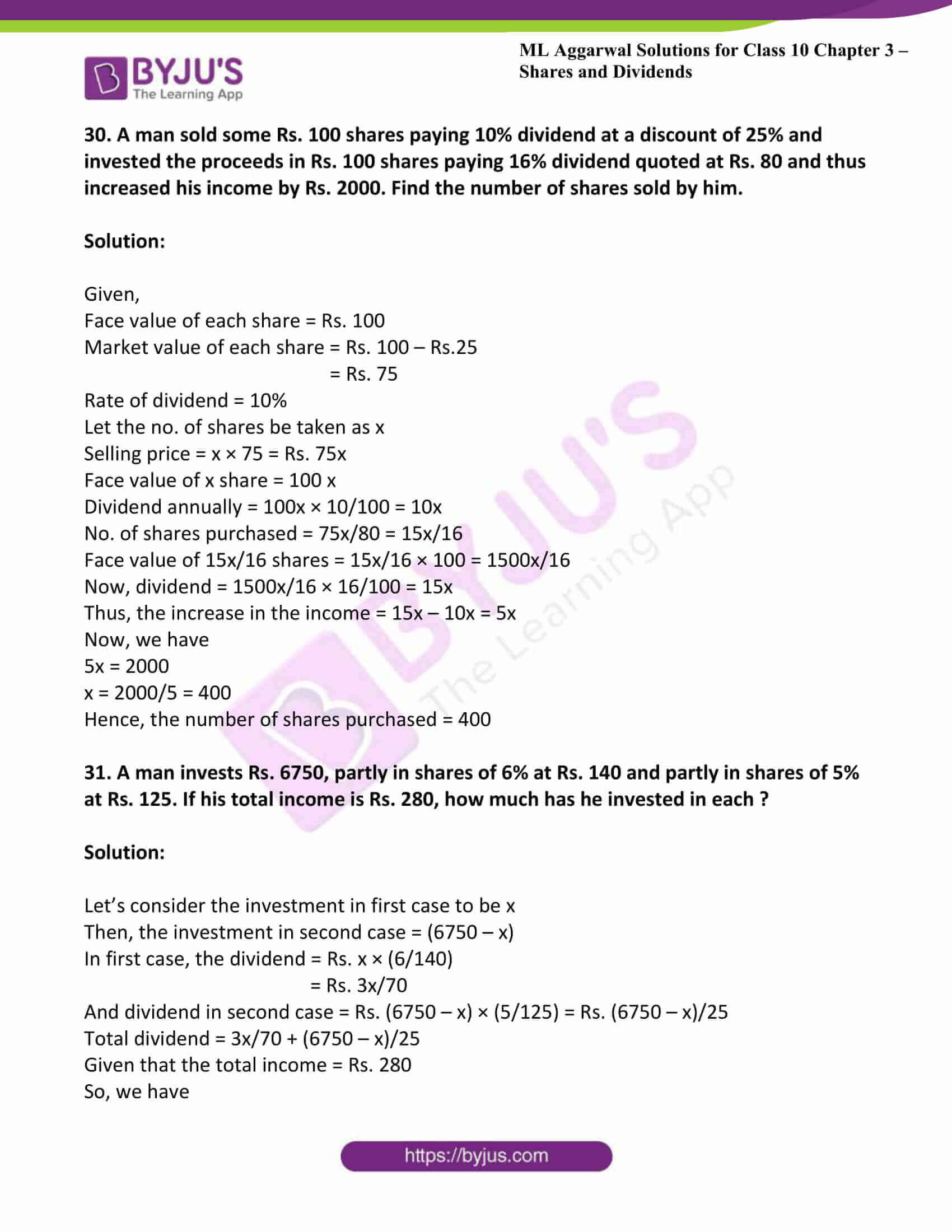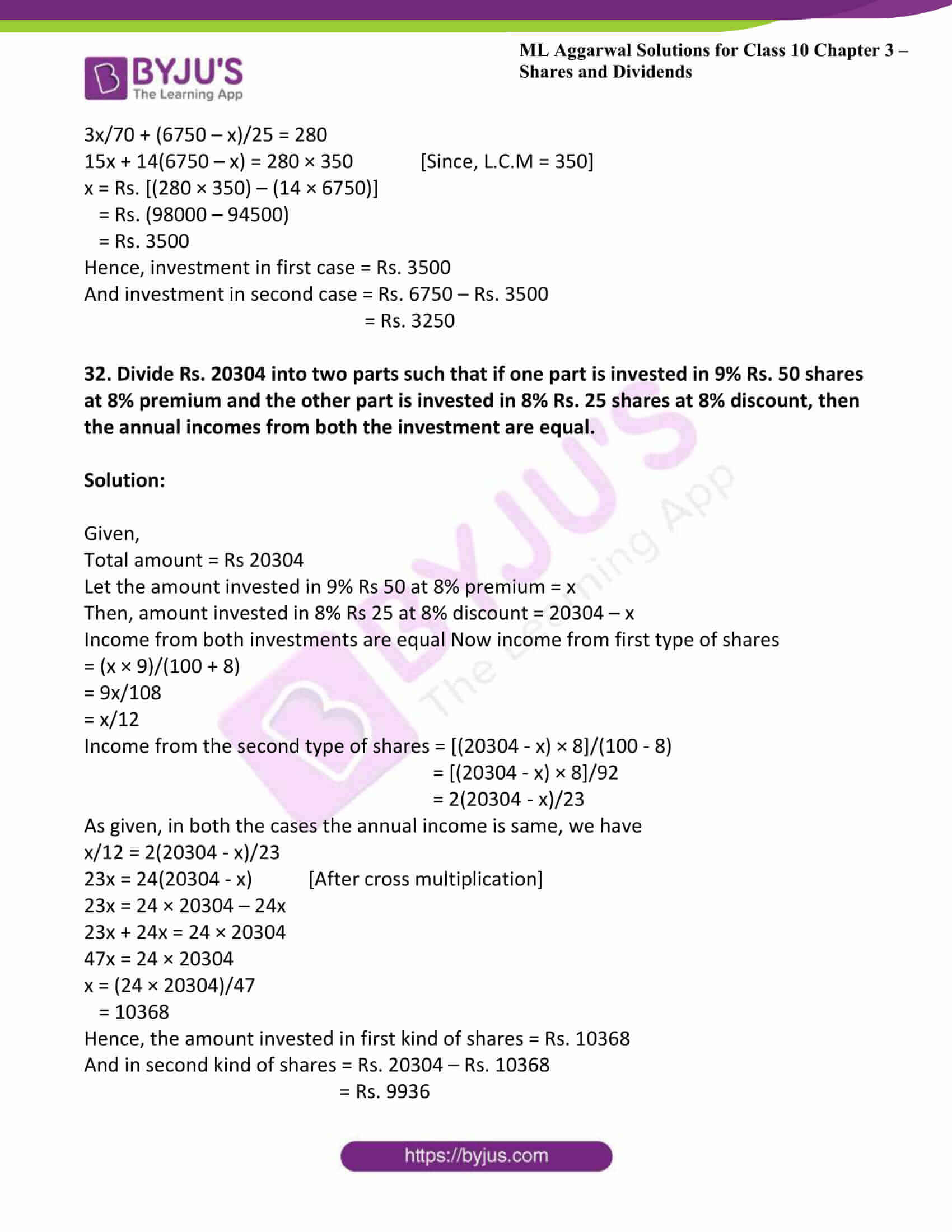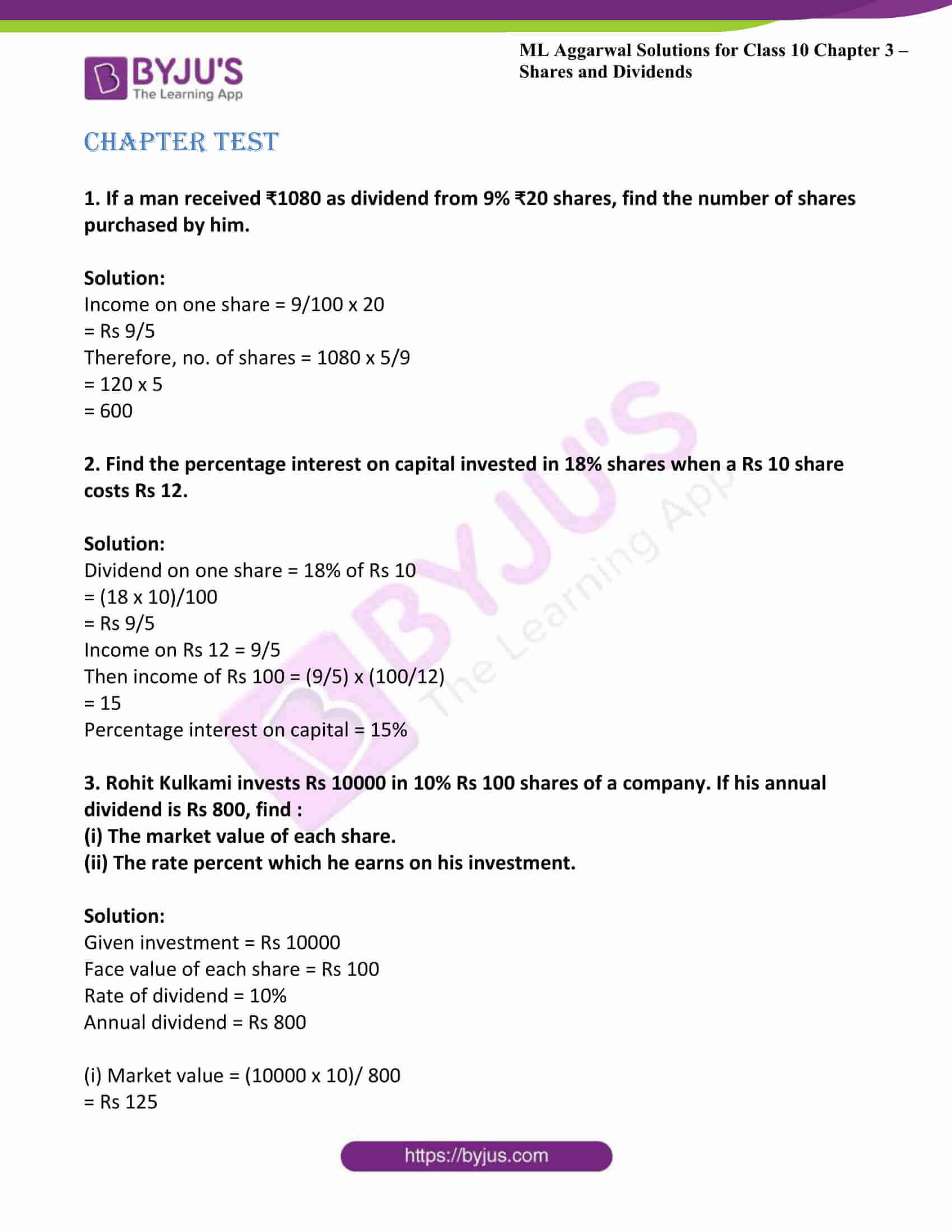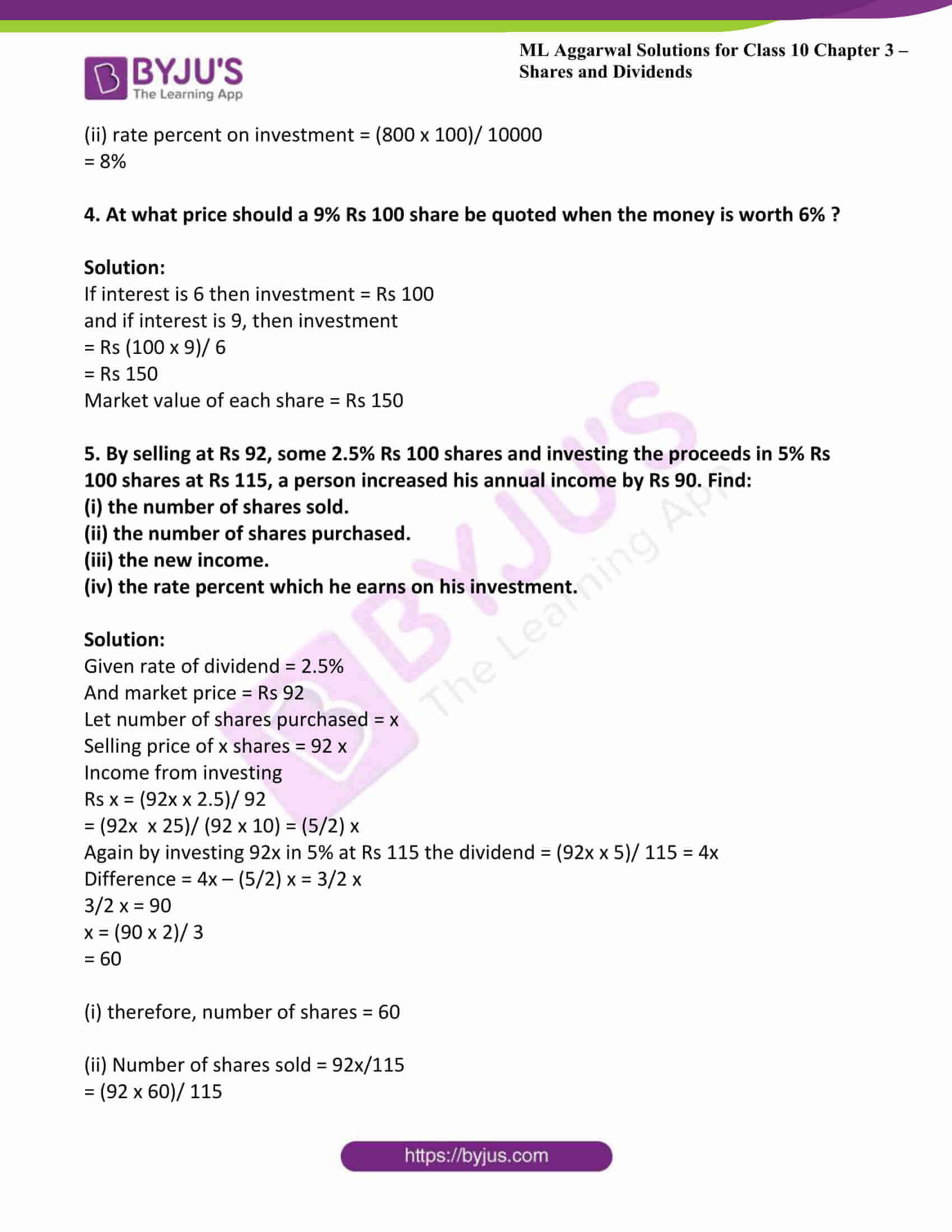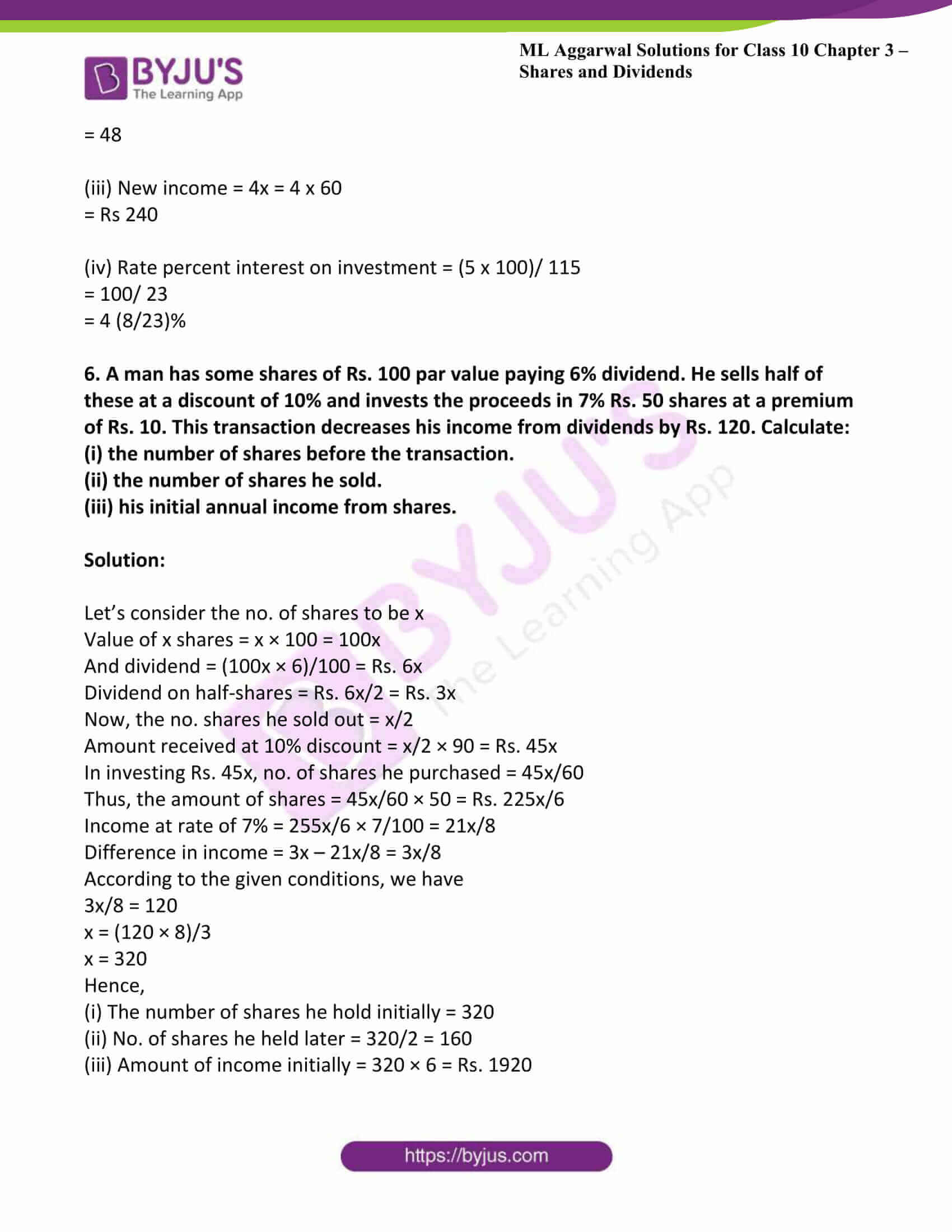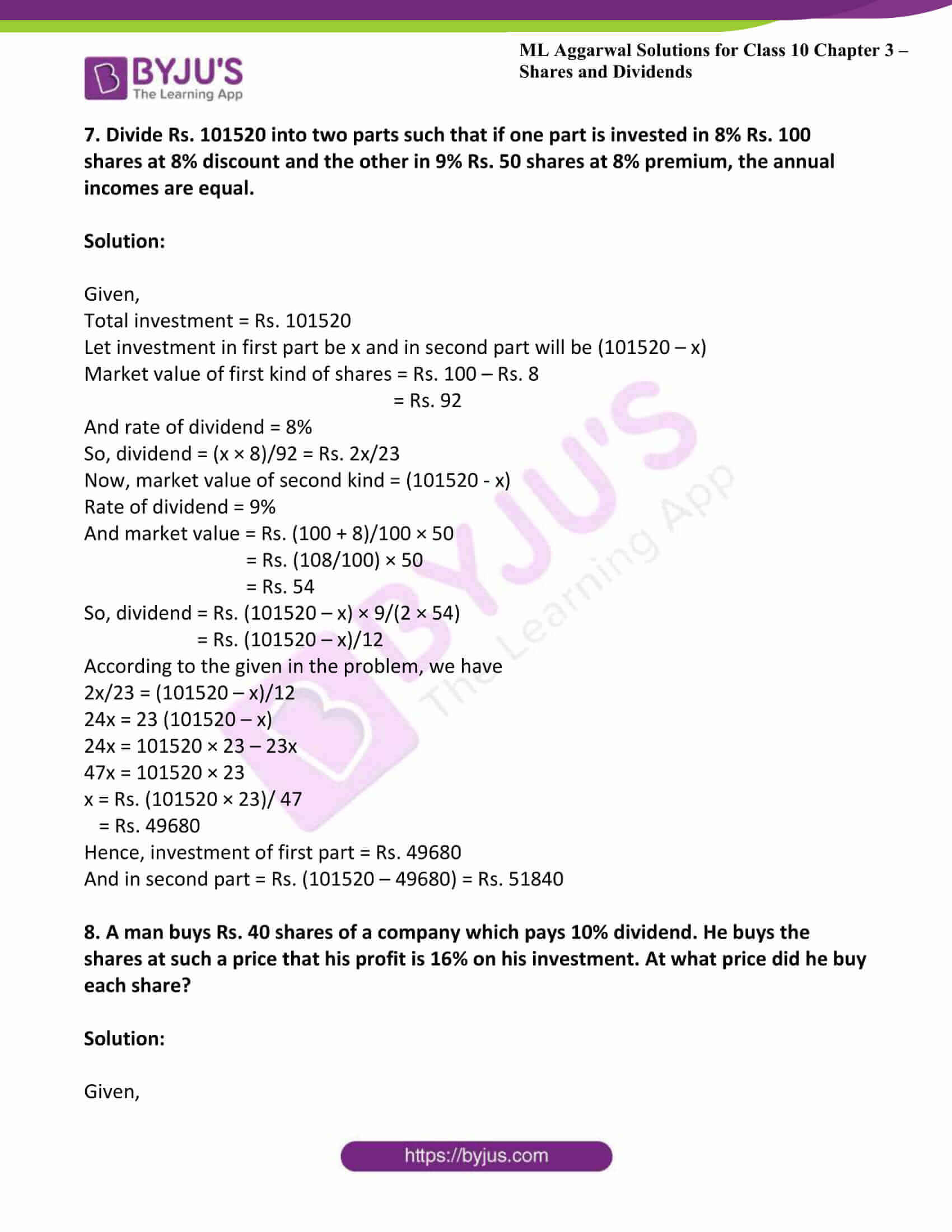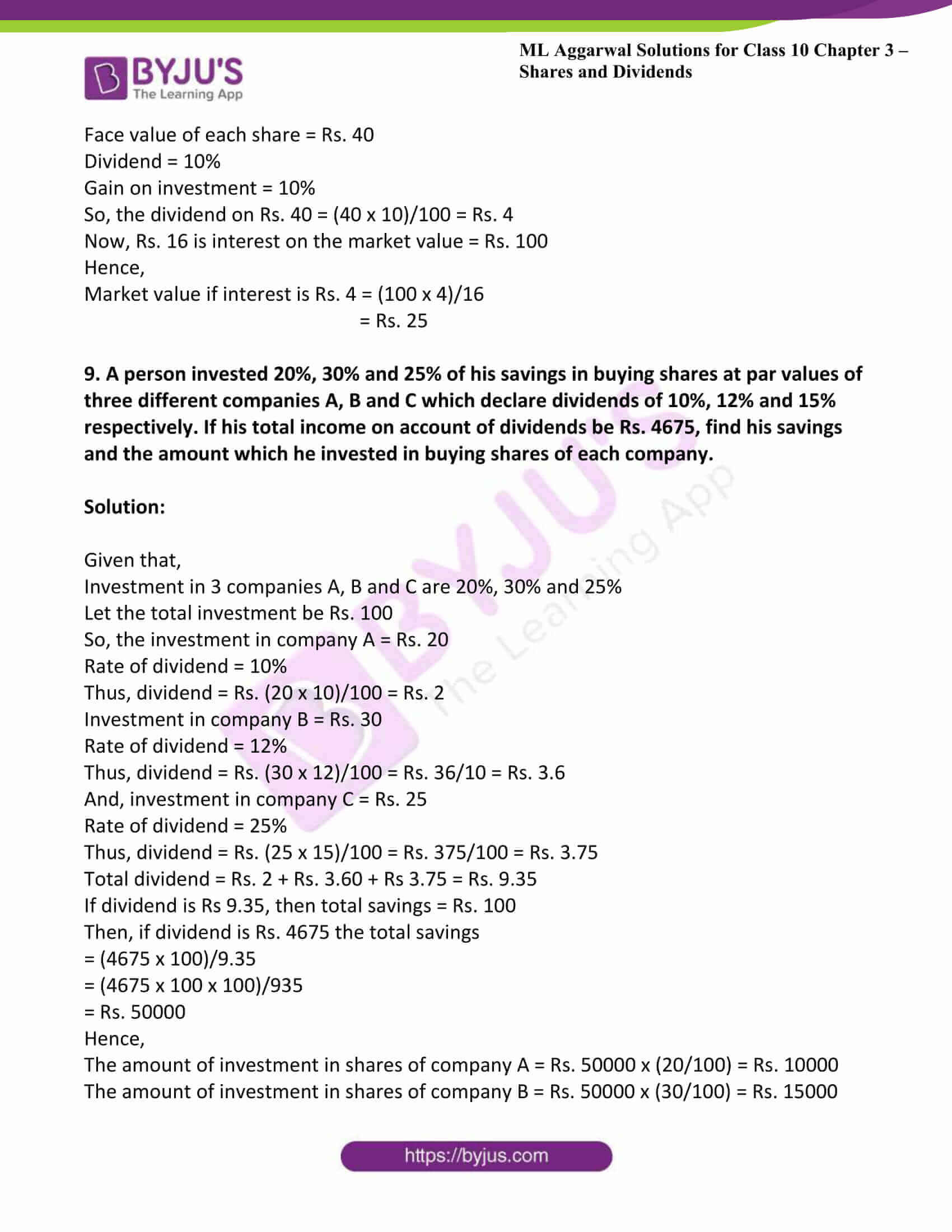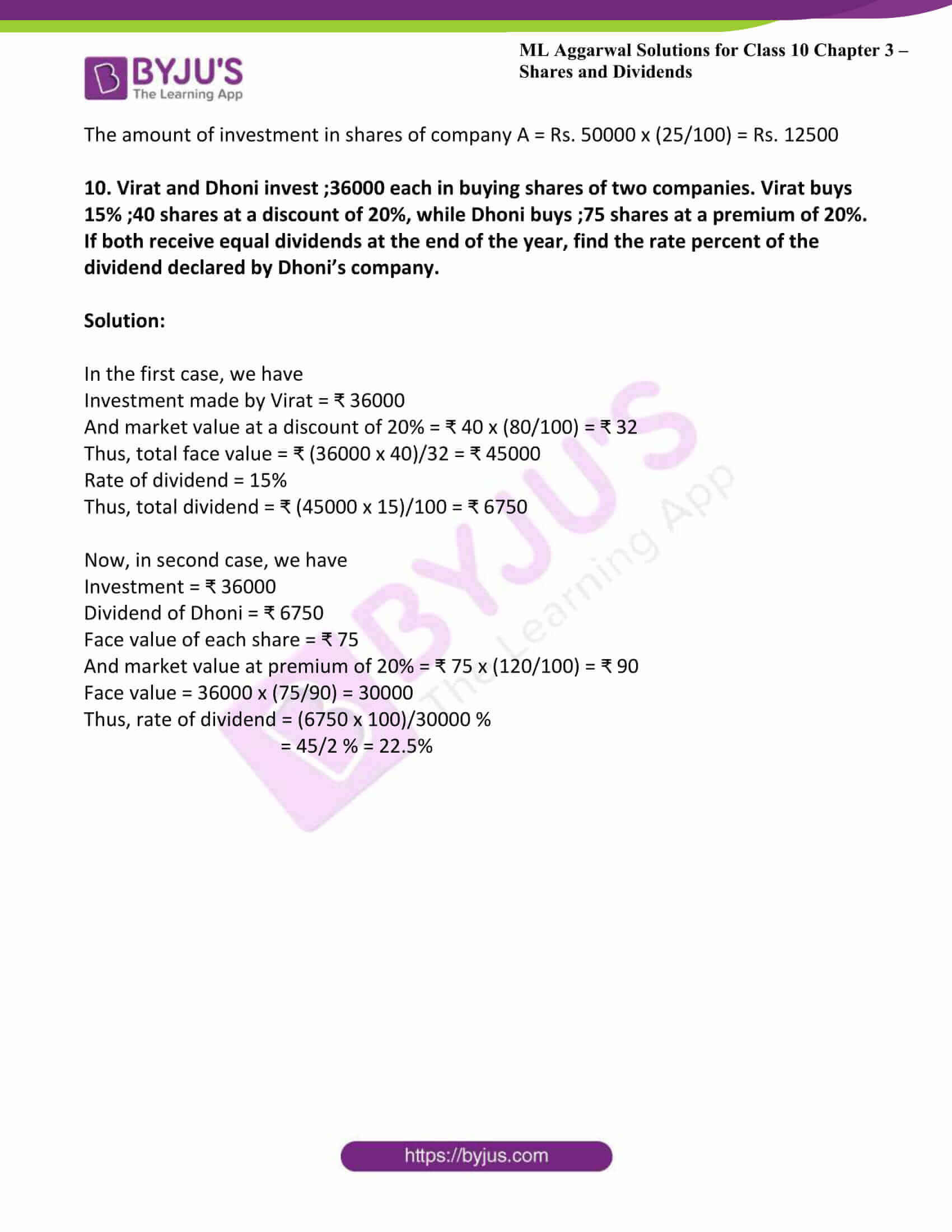### Access answers to ML Aggarwal Solutions for Class 10 Maths Chapter 3 – Shares and Dividends

Exercise 3

1. Find the dividends received on 60 shares of Rs 20 each if 9% dividend is declared.

Solution:

Given value of shares = Rs. 20

Therefore the value of 60 shares = Rs. 20 × 60

= Rs. 1200

Given that rate of dividend = 9%

Therefore total dividend = Rs. 1200 × 9%

= 1200 × (9/100)

= Rs 108

2. A company declares 8 percent dividend to the share holders. If a man receives a Rs. 2840 as his dividend, find the nominal value of his shares.

Solution:

Given that rate of dividend = 8%

Also given that amount of dividend = Rs. 2840

Therefore nominal value of shares = (2840 × 100)/8

= Rs. 35500

3. A man buys 200 ten-rupee shares at Rs. 12.50 each and receives a dividend of 8%. Find the amount invested by him and the dividend received by him in cash.

Solution:

Given face value of shares = Rs. 10

Number of shares = 200

Therefore face value of 200 shares = 10 × 200

= Rs. 2000

Now, amount invested for the purchase of 200 shares at the rate of Rs. 12.50 each

= 12.50 × 200

= Rs. 2500

Given that rate of dividend = 8%

Therefore total amount of dividend = (2000 × 8)/100

= Rs. 160

4. Find the market price of 5% Rs 100 share when a person gets a dividend of Rs 65 by investing Rs. 1430.

Solution:

Given amount of dividend = Rs. 65

Also given that rate of dividend = 5%

Therefore total face value = (65 × 100)/5

= Rs. 1300

If face value is Rs. 1300, then market value = Rs. 140

If face value is Rs. 100, then market value = (1430 × 100)/ 1300

= Rs. 110

5. Salman buys 50 shares of face value Rs 100 available at Rs 132.

(i) what is his investment?

(ii) If the dividend is 7.5% p.a., what will be his annual income?

(iii) If he wants to increase his annual income by Rs 150, how many extra shares should he buy?

Solution:

Given face value = Rs 100

(i) Given that market value = Rs 132

And number of shares = 50

Therefore investment = number of shares × market value

= 50 × 132

= Rs 6600

(ii) We have income per share = 7.5% of face value

= (75/ 10 × 100) × 100

= Rs. 7.5

Therefore annual income = 7.5 × 50

= Rs 375

(iii) Therefore new annual income = 375 + 150 = Rs 525

Therefore number of shares = 525/7.5 = 70

Therefore, number of extra share to be increased = 70 – 50

= 20

6. A lady holds 1800, Rs. 100 shares of a company that pays 15% dividend annually. Calculate her annual dividend. If she had bought these shares at 40% premium, what percentage return does she get on her investment? Give your answer to the nearest integer.

Solution:

Given total number of shares = 1800

Nominal value of each share = Rs. 100

And rate o dividend = 15%

Total face value of 1800 shares = 100 × 1800

= Rs. 180000

Therefore total dividend = 180000 × 15/100

= Rs. 2700

Therefore market value of each share = 100 + 40 = Rs. 140

Now the total investment = 140 × 1800

= Rs. 252000

Therefore percentage on his return

= (27000 × 100)/ 252000

= 10.7%

In integers 11%

7. What sum should a person invest in Rs 25 shares, selling at Rs 36, obtain an income of Rs 720, if the dividend declared is 12%? Also find the percentage return on his income.

Solution:

Given nominal value of each share = Rs. 25

Market value of each share = Rs. 36

Total income = Rs 720

Rate of dividend = 12%

Therefore total nominal value = (100 × 720)/ 12

= Rs 6000

Number of shares = 6000/25

= 240

Total investment = 240 × 36

= Rs 8640

Now, percentage return = (720 × 100)/ 8640

= 8.3%

8. Ashok invests Rs 26400 on 12% Rs 25 shares of a company. If he receives a dividend of Rs 2475, find:

(i) The number of shares he bought.

(ii) The market value of each share.

Solution:

Given investment = Rs 26400

Face value of the each share = Rs 25

Rate of dividend = 12%

Total dividend = Rs 2475

We know that, dividend earned = market price of share × number of shares × r/100

(i) Therefore number of shares = (2475/12) × (100/25)

= 825 shares

(ii) Market value of each share = (26400/825)

= Rs 32

9. A man invests ₹ 4500 in shares of a company which is paying 7.5% dividend. If ₹ 100 shares are available at a discount of 10%, find

(i) the number of shares he purchases.

(ii) his annual income.

Solution:

Given,

Investment = ₹ 4500

Face value of each share = ₹ 100

Discount = 10% and rate of dividend = 7.5%

The market price of each share = ₹ (100 – 25) = ₹ 75

(i) The number of shares he purchases = 4500/75 = 60

(ii) Dividend = ₹ 7.5% of (60 x 100)

= ₹ 450

Hence, his annual income will be ₹ 450.

10. Amit kumar invests Rs 36000 in buying Rs 100 shares at Rs 20 premium. The dividend is 15% per annum. Find:

(i) The number of shares he buys

(ii) His yearly dividend

(iii) The percentage return on his investment.

Solution:

Given investment = Rs 36000

Face value = Rs 100

Premium = Rs 20 and dividend = 15%

(i) Number of shares = 36000/120

= 300

(ii) Dividend = 15% 0f (100 × 300)

= Rs 4500

(iii) Percentage of return = (4500/36000) × 100

= 450/36

= 12.5%

11. Mr. Tiwari invested Rs 29040 in 15% Rs 100 shares at a premium of 20%. Calculate:

(i) The number of shares bought by Mr. Tiwari

(ii) Mr. Tiwari’s income from the investment

(iii) The percentage return on hid investment.

Solution:

(i) Market value of one share = [(200/100) × 100] + 100

= Rs 120

Number of shares = investment/ market value of one share

= 29040/120

= Rs 242

(ii) Therefore income from investment = 242 × 15

= Rs 3630

(iii) Percentage return on his investment = (dividend/ market value) × 100

= (15/120) × 100

= 12.5%

12. A man buys shares at the par value of Rs 10 yielding 8% dividend at the end of a year. Find the number of shares bought if he receives a dividend of Rs 300.

Solution:

Given face value of each share = Rs 10

Rate of dividend = 8% per annum

Total dividend = Rs 300

Therefore total face value of shares = (300 × 100)/ 8

= Rs 3750

Number of shares = 3750/10

= 375

13. A man invests Rs 8800 on buying shares of face value of rupees hundred each at a premium of 10%. If he earns Rs 1200 at the end of year as dividend, find:

(i) The number of shares he has in this company

(ii) The dividend percentage per share.

Solution:

Given investment = Rs 8800

Face value of each share = Rs 100

Market value of each share = 100 + 10 = Rs 110

Total income = Rs 1200

Therefore total face value = (8800 × 100)/ 110

= Rs 8000

(i) Number of shares = 8000/100

= 80

(ii) Rate of dividend = (1200 × 100)/ 8000

= 15%

14. A man invested Rs 45000 in 15% Rs 100 shares quoted at Rs 125. When the market value of these shares rose to Rs 140, he sold some shares, just enough to raise Rs 8400. Calculate:

(i) The number of shares he still holds.

(ii) The dividend due to him on these shares.

Solution:

Given investment on shares = Rs 45000

Face value of each share = Rs 125

Therefore total number of shares = 45000/125

= 360 shares

Income from sold shares = Rs 8400

Therefore number of shares sold = income from shares/ number of shares sold

= 8400/ 140

= 60

(i) Number of shares he still holds = 300

(ii) Market value of 300 shares = 300 × 140

= Rs 42000

Face value of 300 shares = 300 × 125

= Rs 37500

Difference = Market value – face value

= 42000 – 37500

= Rs 4500

15. Ajay owns 560 shares of a company. The face value of each share is Rs 25. The company declares a dividend 0f 9%. Calculate

(i) The dividend that Ajay will get

(ii) The rate of interest, on his investment if Ajay has paid Rs. 30 for each share.

Solution:

Given number of shares = 560

Face value of each share = Rs 25

Rate of dividend = 9% per annum

Total face value of 560 shares = 25 × 560

= Rs 14000

(i) Amount of dividend = 14000 × (9/100)

= Rs 1260

(ii) Market value of each share = Rs 30

Total investment = 30 × 560

= Rs 16800

Therefore percentage of interest on his investment = (1200 × 100)/ 16800

= 7.5%

16. A company with 10000 shares of nominal value of Rs 100 declares an annual dividend of 8% to the share holders.

(i) Calculate the total amount of dividend paid by the company

(ii) Ramesh bought 90 shares of company at Rs 150 per share.

Calculate the dividend he received and the percentage return on his investment.

Solution:

Given number of shares = 10000

Nominal value of each share = Rs 100

Rate of annual dividend = 8%

Total face value of 10000 shares = 100 × 10000

= Rs 1000000

Dividend = (1000000 × 8)/ 100

= Rs 80000

(ii) Number of shares = 90

Face value of each share = Rs 150

Total face value of 90 shares = 100 × 90

= Rs 9000

Therefore amount of dividend = (9000 × 8)/ 100

= Rs 720

Market value of 90 shares = 90 × 159

= Rs 13500

Therefore rate of interest = (720 × 100)/ (13500 × 1)

= 16/3

= 5.3 %

17. A company with 4000 shares of nominal value of Rs. 110 declares annual dividend of 15%. Calculate :

(i) the total amount of dividend paid by the company,

(ii) the annual income of Shah Rukh who holds 88 shares in the company,

(iii) if he received only 10% on his investment, find the price Shah Rukh paid for each share. (2008)

Solution:

Number of shares = 4000

Nominal (face) value of each share = Rs. 110

Total face value of 4000 shares = Rs. 110
x 4000

= Rs, 440000

Rate of annual dividend = 15%

(i) Amount of dividend = (440000 x 15)/ 100

= Rs 66000

(ii) Number of shares, Shah Rukh has = 88

Face value of 88 shares = 88 x 110

= Rs 9680

Annual dividend = (9680 x 15)/ 100

= Rs 1452

(iii) Rate of annual income on his investment = 10%

His investment = (1452 x 100)/ 10

= Rs 14520

Market value of each share = 14520/88

= Rs 165

18. By investing Rs. 7500 in a company paying 10 percent dividend, an income of Rs. 500 is received. What price is paid for each Rs. 100 share.

Solution:

Given investment = Rs. 7500

Rate of dividend = 10%,

Total income = Rs. 500.

Face value of each share = Rs. 100

Total face value = (100 x 500)/10

= Rs 5000

If face value is Rs. 5000, then investment = Rs. 7500

and if face value is Rs. 100 then market value of each share = (7500 x 100)/ 5000

= Rs 150

19. A man buys 400 ten-rupee shares at a premium of Rs. 2.50 on each share. If the rate of dividend is 8%, Find,

(i) his investment

(iii) yield.

Solution:

Given No. of shares = 400

Face value of each share = Rs. 10

Market value of each share

= Rs. 10 + Rs. 2.50

= Rs. 12.50

Rate of dividend = 8%

Therefore, face value of 400 shares = 10 x 400

= Rs 4000

(i) Total investment = 12.50 x 400

= Rs 5000

(ii) Total dividend = 4000
x (8/100)

= Rs 320

(iii) Yield percent = (320 x 100)/ 5000

= 32/5

= 6.4%

20. A man invests Rs. 10400 in 6% shares at Rs. 104 and Rs. 11440 in 10.4% shares at Rs. 143. How much income would he get in all ?

Solution:

Given In first case; Total investment = Rs. 10400

Rate of dividend = 6%

Market value of each share = Rs. 104

Total dividend = (10400 x 6)/ 104

= Rs 600

In second case, investment = Rs 11440

Rate of dividend = 10.4%

Market value of each share = Rs 143

Therefore, total dividend = (11440 x 10.4)/ 143

= Rs 832

Total dividend from both cases = Rs. 600 + Rs. 832

= Rs. 1432

21. Two companies have shares of 7% at Rs. 116 and 9% at Rs. 145 respectively. In which of the shares would the investment be more profitable ?

Solution:

Let the investment in each case = Rs. 116 x 145

Dividend in first case,

= (116 x 145 x 7)/ 116

= Rs 1015

Dividend in second case

= (116 x 145 x 9)/ 145

= Rs 1044

From the above it is clear that the second type of shares that is 9% at Rs 145 are more profitable.

22. Which is better investment : 6% Rs. 100 shares at Rs. 120 or 8% Rs. 10 shares at Rs. 15

Solution:

Let the investment in each case = Rs. 120 In the fist case,

Dividend on Rs. 120 = Rs. 6

In second case, Dividend on Rs. 10

= (8 x 10)/ 100

= 0.8

Now dividend on Rs 15 = 0.8

Then dividend on Rs 120 = (0.8 x 120)/ 15

= Rs 6.4

From the above it is clear that the second type of shares that is 8% at Rs 10shares at 15 is more profitable.

23. A man invests Rs 10080 in 6% hundred- rupee shares at Rs. 112. Find his annual income. When the shares fall to Rs. 96 he sells out the shares and invests the proceeds in 10% ten-rupee shares at Rs. 8. Find the change in his annual income.

Solution:

Given Investment = Rs. 10080

Face value of each share = Rs. 100

Market value of each share = Rs. 112

Rate of dividend = 6%

Total income for the year

= (10080 x 6)/ 112

= Rs 540

Number of shares = 10080/112

= 90

Selling price of 90 shares at the rate of Rs 96 each = 90 x 96

= Rs 8640

Rate of dividend in new shares = 10%

Face value of each share = Rs 10

Market value of each share = Rs 8

Number of shares = 8640/8 = 1080

Face value of 1080 shares = 1080 x 10

= Rs 10800

Dividend = (10800 x 10)/100

= Rs 1080

Difference in income = 1080 – 540

= Rs 540 more

24. Sachin invests ₹ 8500 in 10% ₹ 100 shares at ₹ 170. He sells the shares when the price of each share rises by ₹ 30. He invests the proceeds in 12% ₹ 100 shares at ₹ 125. Find

(i) the sale proceeds.

(ii) the number of ₹ 125 shares he buys.

(iii) the change in his annual income.

Solution:

Given,

Investment = ₹ 8500

Face value of each share = ₹ 100

Market value of each share = ₹ 170

Rate of dividend = 10%

Total income for the year

= ₹ (8500 x 10)/170

= ₹ 500

And, the number of shares = 8500/170 = 50

Selling price of 50 shares at the rate of ₹ (170 + 30) each = ₹ 50 x 200

= ₹ 10000

(i) The sale proceeds = ₹ 10000

Rate of dividend in new shares = 12%

Face value of each share = Rs 100

Market value of each share = Rs 125

(ii) Number of shares = 10000/125 = 80

Face value of 80 shares = ₹ 80 x 100

= ₹ 8000

Dividend = ₹ (8000 x 12)/100

= ₹ 960

(iii) Hence, the change in his annual income = ₹ (960 – 500)

= ₹ 460 more

25. A person invests Rs. 4368 and buys certain hundred-rupee shares at 91. He sells out shares worth Rs. 2400 when they have t risen to 95 and the remainder when they have fallen to 85. Find the gain or loss on the total transaction.

Solution:

Given Investment = Rs. 4368

Market value of each share = Rs. 91

Face value of each share = Rs 100

Therefore, number of shares = 4368/91

= 48

Face value of 24 shares = 24 x 100

= Rs 2400

Sale price of shares worth Rs 2400 = (2400 x 95)/ 100

= Rs 2280

Face value of remaining shares = 24 x 100

= Rs 2400

Sale price of shares of remaining amount = (2400 x 85)/ 100

= Rs 2040

Total amount received = 2280 + 2040

= Rs 4320

Loss = 4368 – 4320

= Rs 48

26. By purchasing Rs. 50 gas shares for Rs. 80 each, a man gets 4% profit on his investment. What rate percent is company paying? What is his dividend if he buys 200 shares?

Solution:

Given market value of each share = Rs 80

Face value of each share = Rs. 50

Interest on investment = 4%

Dividend on Rs 80 = (80 x 4)/ 100

= 32/10

Percent dividend = (32/10) x (100/50)

= 64/10

= 6.4%

Number of shares = 200

Face value of 200 shares = 200 x 50

= Rs 10000

Dividend = Rs 10000 x (6.4/100)

= Rs 640

27. Rs. 100 shares of a company are sold at a discount of Rs. 20. If the return on the investment is 15%. Find the rate of dividend declared.

Solution:

Market value of each shares = 100 – 20 = Rs.80

Interest on investment of Rs. 80 = 15% x 80

= 15/100
x 80

= Rs 12

Dividend on face value of Rs. 100 = Rs. 12

Rate of dividend = 12%.

28. A company declared a dividend of 14%. Find tire market value of Rs. 50 shares if the return on the investment was 10%.

Solution:

Rate of dividend = 14%

Dividend on Rs. 50 = (14 x 50)/ 100

= Rs 7

Now Rs. 10 is interest on the investment of = Rs. 100

Rs. 7 will be the interest on = (100 x 7)/ 10 = Rs. 70

Hence, market value of Rs. 50 shares = Rs. 70

29. A company with 10000 shares of Rs. 100 each, declares an annual dividend of 5%.
(i) What is the total amount of dividend paid by the company ?
(ii) What would be the annual income of a man, who has 72 shares, in the company ?
(iii) If he received only 4% on his investment, find the price he paid for each share.

Solution:

Given,

No. of shares = 10000

Face value of each share = Rs. 100

Rate of dividend = 5%

(i) Total face value of 10000 shares

= Rs. 100 x 10000

= Rs. 1000000

Hence,

Total amount of dividend = Rs. (1000000 × 5)/100

= Rs. 50000

(ii) Income of 72 shares = 72 × 5 = Rs. 360

(iii) Rate of interest on investment = 4%

Hence, market value of each share = Rs. 100/4 × 5

= Rs. 125

30. A man sold some Rs. 100 shares paying 10% dividend at a discount of 25% and invested the proceeds in Rs. 100 shares paying 16% dividend quoted at Rs. 80 and thus increased his income by Rs. 2000. Find the number of shares sold by him.

Solution:

Given,

Face value of each share = Rs. 100

Market value of each share = Rs. 100 – Rs.25

= Rs. 75

Rate of dividend = 10%

Let the no. of shares be taken as x

Selling price = x × 75 = Rs. 75x

Face value of x share = 100 x

Dividend annually = 100x × 10/100 = 10x

No. of shares purchased = 75x/80 = 15x/16

Face value of 15x/16 shares = 15x/16 × 100 = 1500x/16

Now, dividend = 1500x/16 × 16/100 = 15x

Thus, the increase in the income = 15x – 10x = 5x

Now, we have

5x = 2000

x = 2000/5 = 400

Hence, the number of shares purchased = 400

31. A man invests Rs. 6750, partly in shares of 6% at Rs. 140 and partly in shares of 5% at Rs. 125. If his total income is Rs. 280, how much has he invested in each ?

Solution:

Let’s consider the investment in first case to be x

Then, the investment in second case = (6750 – x)

In first case, the dividend = Rs. x × (6/140)

= Rs. 3x/70

And dividend in second case = Rs. (6750 – x) × (5/125) = Rs. (6750 – x)/25

Total dividend = 3x/70 + (6750 – x)/25

Given that the total income = Rs. 280

So, we have

3x/70 + (6750 – x)/25 = 280

15x + 14(6750 – x) = 280 × 350 [Since, L.C.M = 350]

x = Rs. [(280 × 350) – (14 × 6750)]

= Rs. (98000 – 94500)

= Rs. 3500

Hence, investment in first case = Rs. 3500

And investment in second case = Rs. 6750 – Rs. 3500

= Rs. 3250

32. Divide Rs. 20304 into two parts such that if one part is invested in 9% Rs. 50 shares at 8% premium and the other part is invested in 8% Rs. 25 shares at 8% discount, then the annual incomes from both the investment are equal.

Solution:

Given,

Total amount = Rs 20304

Let the amount invested in 9% Rs 50 at 8% premium = x

Then, amount invested in 8% Rs 25 at 8% discount = 20304 – x

Income from both investments are equal Now income from first type of shares

= (x × 9)/(100 + 8)

= 9x/108

= x/12

Income from the second type of shares = [(20304 – x) × 8]/(100 – 8)

= [(20304 – x) × 8]/92

= 2(20304 – x)/23

As given, in both the cases the annual income is same, we have

x/12 = 2(20304 – x)/23

23x = 24(20304 – x) [After cross multiplication]

23x = 24 × 20304 – 24x

23x + 24x = 24 × 20304

47x = 24 × 20304

x = (24 × 20304)/47

= 10368

Hence, the amount invested in first kind of shares = Rs. 10368

And in second kind of shares = Rs. 20304 – Rs. 10368

= Rs. 9936

Chapter test

1. If a man received ₹1080 as dividend from 9% ₹20 shares, find the number of shares purchased by him.

Solution:

Income on one share = 9/100 x 20

= Rs 9/5

Therefore, no. of shares = 1080 x 5/9

= 120 x 5

= 600

2. Find the percentage interest on capital invested in 18% shares when a Rs 10 share costs Rs 12.

Solution:

Dividend on one share = 18% of Rs 10

= (18 x 10)/100

= Rs 9/5

Income on Rs 12 = 9/5

Then income of Rs 100 = (9/5) x (100/12)

= 15

Percentage interest on capital = 15%

3. Rohit Kulkami invests Rs 10000 in 10% Rs 100 shares of a company. If his annual dividend is Rs 800, find :

(i) The market value of each share.

(ii) The rate percent which he earns on his investment.

Solution:

Given investment = Rs 10000

Face value of each share = Rs 100

Rate of dividend = 10%

Annual dividend = Rs 800

(i) Market value = (10000 x 10)/ 800

= Rs 125

(ii) rate percent on investment = (800 x 100)/ 10000

= 8%

4. At what price should a 9% Rs 100 share be quoted when the money is worth 6% ?

Solution:

If interest is 6 then investment = Rs 100

and if interest is 9, then investment

= Rs (100 x 9)/ 6

= Rs 150

Market value of each share = Rs 150

5. By selling at Rs 92, some 2.5% Rs 100 shares and investing the proceeds in 5% Rs 100 shares at Rs 115, a person increased his annual income by Rs 90. Find:

(i) the number of shares sold.

(ii) the number of shares purchased.

(iii) the new income.

(iv) the rate percent which he earns on his investment.

Solution:

Given rate of dividend = 2.5%

And market price = Rs 92

Let number of shares purchased = x

Selling price of x shares = 92 x

Income from investing

Rs x = (92x x 2.5)/ 92

= (92x x 25)/ (92 x 10) = (5/2) x

Again by investing 92x in 5% at Rs 115 the dividend = (92x x 5)/ 115 = 4x

Difference = 4x – (5/2) x = 3/2 x

3/2 x = 90

x = (90 x 2)/ 3

= 60

(i) therefore, number of shares = 60

(ii) Number of shares sold = 92x/115

= (92 x 60)/ 115

= 48

(iii) New income = 4x = 4 x 60

= Rs 240

(iv) Rate percent interest on investment = (5 x 100)/ 115

= 100/ 23

= 4 (8/23)%

6. A man has some shares of Rs. 100 par value paying 6% dividend. He sells half of these at a discount of 10% and invests the proceeds in 7% Rs. 50 shares at a premium of Rs. 10. This transaction decreases his income from dividends by Rs. 120. Calculate:
(i) the number of shares before the transaction.
(ii) the number of shares he sold.
(iii) his initial annual income from shares.

Solution:

Let’s consider the no. of shares to be x

Value of x shares = x × 100 = 100x

And dividend = (100x × 6)/100 = Rs. 6x

Dividend on half-shares = Rs. 6x/2 = Rs. 3x

Now, the no. shares he sold out = x/2

Amount received at 10% discount = x/2 × 90 = Rs. 45x

In investing Rs. 45x, no. of shares he purchased = 45x/60

Thus, the amount of shares = 45x/60 × 50 = Rs. 225x/6

Income at rate of 7% = 255x/6 × 7/100 = 21x/8

Difference in income = 3x – 21x/8 = 3x/8

According to the given conditions, we have

3x/8 = 120

x = (120 × 8)/3

x = 320

Hence,

(i) The number of shares he hold initially = 320

(ii) No. of shares he held later = 320/2 = 160

(iii) Amount of income initially = 320 × 6 = Rs. 1920

7. Divide Rs. 101520 into two parts such that if one part is invested in 8% Rs. 100 shares at 8% discount and the other in 9% Rs. 50 shares at 8% premium, the annual incomes are equal.

Solution:

Given,

Total investment = Rs. 101520

Let investment in first part be x and in second part will be (101520 – x)

Market value of first kind of shares = Rs. 100 – Rs. 8

= Rs. 92

And rate of dividend = 8%

So, dividend = (x × 8)/92 = Rs. 2x/23

Now, market value of second kind = (101520 – x)

Rate of dividend = 9%

And market value = Rs. (100 + 8)/100 × 50

= Rs. (108/100) × 50

= Rs. 54

So, dividend = Rs. (101520 – x) × 9/(2 × 54)

= Rs. (101520 – x)/12

According to the given in the problem, we have

2x/23 = (101520 – x)/12

24x = 23 (101520 – x)

24x = 101520 × 23 – 23x

47x = 101520 × 23

x = Rs. (101520 × 23)/ 47

= Rs. 49680

Hence, investment of first part = Rs. 49680

And in second part = Rs. (101520 – 49680) = Rs. 51840

8. A man buys Rs. 40 shares of a company which pays 10% dividend. He buys the shares at such a price that his profit is 16% on his investment. At what price did he buy each share?

Solution:

Given,

Face value of each share = Rs. 40

Dividend = 10%

Gain on investment = 10%

So, the dividend on Rs. 40 = (40 x 10)/100 = Rs. 4

Now, Rs. 16 is interest on the market value = Rs. 100

Hence,

Market value if interest is Rs. 4 = (100 x 4)/16

= Rs. 25

9. A person invested 20%, 30% and 25% of his savings in buying shares at par values of three different companies A, B and C which declare dividends of 10%, 12% and 15% respectively. If his total income on account of dividends be Rs. 4675, find his savings and the amount which he invested in buying shares of each company.

Solution:

Given that,

Investment in 3 companies A, B and C are 20%, 30% and 25%

Let the total investment be Rs. 100

So, the investment in company A = Rs. 20

Rate of dividend = 10%

Thus, dividend = Rs. (20 x 10)/100 = Rs. 2

Investment in company B = Rs. 30

Rate of dividend = 12%

Thus, dividend = Rs. (30 x 12)/100 = Rs. 36/10 = Rs. 3.6

And, investment in company C = Rs. 25

Rate of dividend = 25%

Thus, dividend = Rs. (25 x 15)/100 = Rs. 375/100 = Rs. 3.75

Total dividend = Rs. 2 + Rs. 3.60 + Rs 3.75 = Rs. 9.35

If dividend is Rs 9.35, then total savings = Rs. 100

Then, if dividend is Rs. 4675 the total savings

= (4675 x 100)/9.35

= (4675 x 100 x 100)/935

= Rs. 50000

Hence,

The amount of investment in shares of company A = Rs. 50000 x (20/100) = Rs. 10000

The amount of investment in shares of company B = Rs. 50000 x (30/100) = Rs. 15000

The amount of investment in shares of company A = Rs. 50000 x (25/100) = Rs. 12500

10. Virat and Dhoni invest ;36000 each in buying shares of two companies. Virat buys 15% ;40 shares at a discount of 20%, while Dhoni buys ;75 shares at a premium of 20%. If both receive equal dividends at the end of the year, find the rate percent of the dividend declared by Dhoni’s company.

Solution:

In the first case, we have

Investment made by Virat = ₹ 36000

And market value at a discount of 20% = ₹ 40 x (80/100) = ₹ 32

Thus, total face value = ₹ (36000 x 40)/32 = ₹ 45000

Rate of dividend = 15%

Thus, total dividend = ₹ (45000 x 15)/100 = ₹ 6750

Now, in second case, we have

Investment = ₹ 36000

Dividend of Dhoni = ₹ 6750

Face value of each share = ₹ 75

And market value at premium of 20% = ₹ 75 x (120/100) = ₹ 90

Face value = 36000 x (75/90) = 30000

Thus, rate of dividend = (6750 x 100)/30000 %

= 45/2 % = 22.5%